用c++做图像处理_c++ 图像 处理 dev c++ - CSDN
• 1、图像读取2、图像显示3、获取图像的属性4、图像遍历的几种方式
    使用C++开发图像处理算法时，不需要去调用OpenCV的内置算法函数，主要是利用OpenCV完成图像文件的输入、输出以及自动内存管理（重点）。所以，只要需要掌握一些简单的OpenCV的操作即可。

1、图像读取

CV_EXPORTS_W Mat imread( const String& filename, int flags = IMREAD_COLOR );

filename参数为图像文件的路径，可以是相对路径，也可以是绝对路径；flags参数为图像文件解析的方式，支持的方式有如下：

示例代码：

	//不做任何转化进行读取

//彩色模式进行读取

//灰度模式进行读取
cv::imshow("GRAYSCALE", matGrayScale);

运行结果：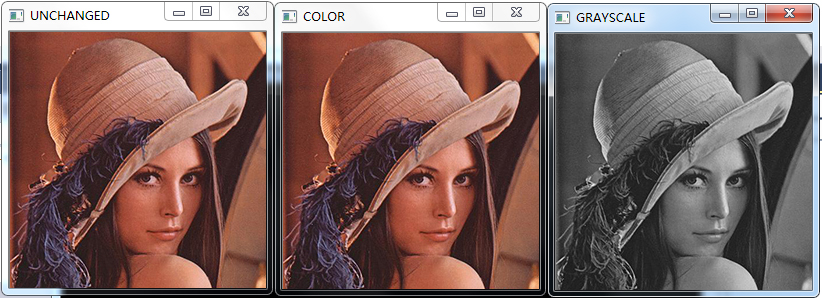2、图像显示

OpenCV的图像显示函数为imshow，函数原型如下：

CV_EXPORTS_W void imshow(const String& winname, InputArray mat);

winname参数表示显示图像窗口的名称（任意字符），mat参数表示需要显示的图像。对于这个函数，需要注意的是（特别是新手），imshow函数只支持8位灰度图像、8位彩色图像和32位灰度图像（像素值范围0-1），具体原因大家可以自行百度关键词【显示器灰度等级】。

3、获取图像的属性

图像的常用属性有图像的宽度、高度、数据缓存区指针（图像像素存储地址）、图像的通道数、深度。示例代码：

       cv::Mat matUnchanged = cv::imread("./lena.jpg", cv::IMREAD_UNCHANGED);
//宽度
std::cout << "宽度："<<matUnchanged.cols << std::endl;
//高度
std::cout << "高度：" << matUnchanged.rows << std::endl;
//数据缓存区指针
unsigned char* pData = matUnchanged.data;
std::cout << "数据地址：" << &pData << std::endl;
//通道数
std::cout << "通道数：" << matUnchanged.channels() << std::endl;
//深度
//elemSize函数返回的是一个像素占用的字节数
std::cout << "深度：" << matUnchanged.elemSize() / matUnchanged.channels() * 8 << std::endl;
cv::imshow("UNCHANGED", matUnchanged);
cv::waitKey(0);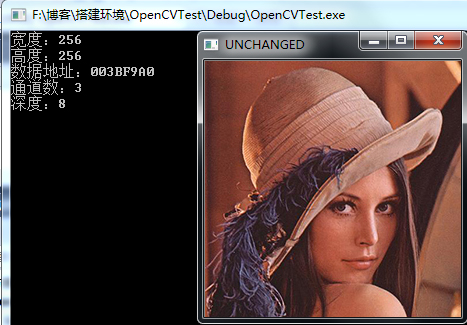4、图像遍历的几种方式

作为示例，读取一副RGB彩色图像，将每个像素的R值置0作为测试。（在Windows下，RGB存储的顺序为BGR）

（1）、使用OpenCV的at成员函数

OpenCV提供了便利的访问图像数据的接口，at函数原型：

template<typename _Tp> inline _Tp& Mat::at(int i0, int i1)

参数i0为行号，i1为列号；模板参数_Tp常用类型如下：

图像类型
_Tp参数
单通道灰度
unsigned char
三通道彩色（8位）
cv::Vec3b
16位灰度

unsigned short

32位浮点型
float
双通道32位
cv::Vec2f

示例代码：

for (size_t r = 0; r < matUnchanged.rows; r++)//行
for (size_t c = 0; c < matUnchanged.cols; c++)//列
{
cv::Vec3b& bgr = matUnchanged.at<cv::Vec3b>(r, c);
bgr = 0;
}

（2）、使用指针

for (size_t r = 0; r < matUnchanged.rows; r++)//行
{
//step返回图像一行的字节数
unsigned char* pRow = matUnchanged.data + r * matUnchanged.step;//计算图像行首指针
for (size_t c = 0; c < matUnchanged.cols; c++)//列
{
pRow[3 * c + 2] = 0;
}
}

（3）、行首指针存储法

//存储图像行首指针
std::vector<unsigned char*> rowPtr(matUnchanged.rows);
for (size_t r = 0; r < matUnchanged.rows; r++)
rowPtr[r] = matUnchanged.data + r * matUnchanged.step;

//遍历图像
for (size_t r = 0; r < matUnchanged.rows; r++)//行
for (size_t c = 0; c < matUnchanged.cols; c++)//列
{
rowPtr[r][c * 3 + 2] = 0;
}

访问图像数据的方式有很多，这里只列出几种常用的方式，考虑到算法的效率问题，通常使用指针对数据进行访问。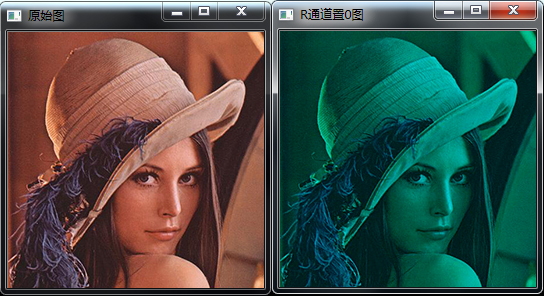技术交流合作QQ：3355138068

展开全文• 查资料时看到的一篇博文，转下来先，原文：http://gaojinpeng1129.blog.163.com/blog/static/141659068201321194552695/ [cpp] view plain copy .../*函数名称：MakegGray() *
查资料时看到的一篇博文，转下来先，原文：http://gaojinpeng1129.blog.163.com/blog/static/141659068201321194552695/

[cpp] view
plain copy

/*函数名称：MakegGray()                                        */
/*函数类型：void                                               */
/*功能：真彩色转化成256色灰度图像。                            */
/***************************************************************/
void MakeColorDib::MakegGray()    //灰度变化
{
BYTE *p_data;     //原图数据区指针
int wide,height,DibWidth;    //原图长、宽、字节宽
p_data=this->GetData ();   //取得原图的数据区指针
wide=this->GetWidth ();   //取得原图的数据区宽度
height=this->GetHeight ();   //取得原图的数据区高度
DibWidth=this->GetDibWidthBytes();   //取得原图的每行字节数
for(int j=0;j<height;j++) // 每行
for(int i=0;i<DibWidth;i+=3) // 每列
{
BYTE* pbyBlue = p_data++;   //得到蓝色值
BYTE* pbyGreen = p_data++;  //得到绿色值
BYTE* pbyRed = p_data++;    //得到红色值
BYTE r = *pbyRed;
BYTE g = *pbyGreen;
BYTE b = *pbyBlue;
//取到原r,g,b中的最大值作为像素三分量值的新值
int gray=0;
if(r>g)
gray=r;
else
gray=g;
if(gray<b)
gray=b;
*pbyBlue = gray;     //将取到的最大值赋给像素的蓝分量
*pbyGreen = gray;    //将取到的最大值赋给像素的绿分量
*pbyRed = gray;      //将取到的最大值赋给像素的红分量
}
}

/***************************************************************/
/*函数名称：LightAlter(int m_Light)                            */
/*函数类型：void                                               */
/*参数：int m_Light，用户给定的阈值                            */
/*功能：对图像使用阈值法进行亮度调整。                         */
/***************************************************************/
void MakeColorDib::LightAlter(int m_Light)    //亮度调整
{
BYTE *p_data;     //原图数据区指针
int wide,height,DibWidth;    //原图长、宽、字节宽
p_data=this->GetData ();   //取得原图的数据区指针
wide=this->GetWidth ();  //取得原图的数据区宽度
height=this->GetHeight ();    //取得原图的数据区高度
DibWidth=this->GetDibWidthBytes();   //取得原图的每行字节数
for(int j=0;j<height;j++) // 每行
for(int i=0;i<DibWidth;i++) // 每列
{
int a=0;
a=int(*p_data*m_Light/100);   //调整当前点的亮度
*p_data=a;
//判断范围，取得合理的值
if(a<0)
*p_data=0;
if(a>255)
*p_data=255;
p_data++;   //指向下一指针
}
}

/***************************************************************/
/*函数名称：LightReverse()                                     */
/*函数类型：void                                               */
/*功能：图像的亮度取反。                                       */
/***************************************************************/
void MakeColorDib::LightReverse()    //亮度取反
{
BYTE *p_data;     //原图数据区指针
int wide,height,DibWidth;    //原图长、宽、字节宽
p_data=this->GetData ();   //取得原图的数据区指针
wide=this->GetWidth ();  //取得原图的数据区宽度
height=this->GetHeight ();   //取得原图的数据区高度
DibWidth=this->GetDibWidthBytes();   //取得原图的每行字节数
for(int j=0;j<height;j++) // 每行
for(int i=0;i<DibWidth;i++) // 每列
{
int a=*p_data;   //取得当前点的值
*p_data=255-a;    //取反
p_data++;  //指向下一指针
}
}

/***************************************************************/
/*函数名称：ContrastAlter(int m_Increment)                     */
/*函数类型：void                                               */
/*参数：int m_Increment，用户给定的阈值                        */
/*功能：对图像使用阈值法调整对比度处理。                       */
/***************************************************************/
void MakeColorDib::ContrastAlter(int m_Increment)   ///对比度处理
{
int nHigh = 255 - m_Increment;
//对于极端情况加以处理
if(nHigh < m_Increment)
{
nHigh = 127;
m_Increment = 120;
}
if(m_Increment < -127)
m_Increment = -120;
//扩展或压缩区间的长度
int nStretch = 255;
if(m_Increment >= 0)
nStretch = 255 - 2 * m_Increment;
else
nStretch = 255 + 2 * m_Increment;
BYTE *p_data;     //原图数据区指针
int wide,height,DibWidth;    //原图长、宽、字节宽
p_data=this->GetData ();   //取得原图的数据区指针
wide=this->GetWidth ();  //取得原图的数据区宽度
height=this->GetHeight ();   //取得原图的数据区高度
DibWidth=this->GetDibWidthBytes();   //取得原图的每行字节数
if(m_Increment >= 0)   // m_Increment>=0时
{
for(int j=0;j<height;j++) // 每行
for(int i=0;i<DibWidth-3;i+=3) // 每列
{
//取得当前点（蓝色）的值，调整
BYTE* pbyBlue = p_data++;
if(*pbyBlue<=m_Increment)
*pbyBlue=0;
else if(*pbyBlue>nHigh)
*pbyBlue=255;
else
*pbyBlue=(BYTE)((((int)*pbyBlue - m_Increment) * 255) / nStretch );
//取得当前点（绿色）的值，调整
BYTE* pbyGreen = p_data++;
if(*pbyGreen<=m_Increment)
*pbyGreen=0;
else if(*pbyGreen>nHigh)
*pbyGreen=255;
else
*pbyGreen=(BYTE)((((int)*pbyGreen - m_Increment) * 255) / nStretch );
//取得当前点（红色）的值，调整
BYTE* pbyRed = p_data++;
if(*pbyRed<=m_Increment)
*pbyRed=0;
else if(*pbyRed>nHigh)
*pbyRed=255;
else
*pbyRed=(BYTE)((((int)*pbyRed - m_Increment) * 255) / nStretch );
}

}
else  // m_Increment < 0 时
{
for(int j=0;j<height;j++)
for(int i=0;i<DibWidth-3;i+=3)
{ //取得当前点（蓝色）的值，调整
BYTE* pbyBlue = p_data++;
*pbyBlue = (BYTE)((((int)(*pbyBlue) * nStretch) / 255) - m_Increment);
//取得当前点（红色）的值，调整
BYTE* pbyGreen = p_data++;
*pbyGreen = (BYTE)((((int)(*pbyGreen) * nStretch) / 255) - m_Increment);
//取得当前点（红色）的值，调整
BYTE* pbyRed = p_data++;
*pbyRed = (BYTE)((((int)(*pbyRed) * nStretch) / 255) - m_Increment);
}
}
}

/***************************************************************/
/*函数名称：Exposal()                                          */
/*函数类型：void                                               */
/*功能：图像曝光处理。                                         */
/***************************************************************/
void MakeColorDib::Exposal() //曝光处理
{
BYTE *p_data;     //原图数据区指针
int wide,height,DibWidth;    //原图长、宽、字节宽
p_data=this->GetData ();   //取得原图的数据区指针
wide=this->GetWidth ();  //取得原图的数据区宽度
height=this->GetHeight ();   //取得原图的数据区高度
DibWidth=this->GetDibWidthBytes();   //取得原图的每行字节数
for(int j=0;j<height;j++) // 每行
for(int i=0;i<DibWidth;i++) // 每列
{
BYTE* pbydata = p_data++;  //取得当前点的值
BYTE a=*pbydata;   //传给临时变量
*pbydata=(a>128)?a:(255-a);   //调整
}

}

/***************************************************************/
/*函数名称：PaintColor(int m_Red,int m_Green,int m_Blue)       */
/*函数类型：void                                               */
/*参数：int m_Red、m_Green、m_Blue，用户给定的红绿蓝值         */
/*功能：对图像使用阈值法进行着色处理。                         */
/***************************************************************/
void MakeColorDib::PaintColor(int m_Red,int m_Green,int m_Blue) //着色处理
{
BYTE *p_data;     //原图数据区指针
int wide,height,DibWidth;    //原图长、宽、字节宽
p_data=this->GetData ();   //取得原图的数据区指针
wide=this->GetWidth ();  //取得原图的数据区宽度
height=this->GetHeight ();   //取得原图的数据区高度
DibWidth=this->GetDibWidthBytes();   //取得原图的每行字节数
for(int j=0;j<height;j++) // 每行
for(int i=0;i<DibWidth;i+=3) // 每列
{
BYTE* pbyBlue = p_data++; //取得当前点（蓝色）的值
BYTE* pbyGreen = p_data++;  //取得当前点（绿色）的值
BYTE* pbyRed = p_data++;    //取得当前点（红色）的值
BYTE r = *pbyRed;
BYTE g = *pbyGreen;
BYTE b = *pbyBlue;
BYTE gray=(BYTE)(((WORD)r * 59 + (WORD)g * 30 + (WORD)b * 11) / 100);
*pbyBlue = (BYTE)((m_Blue * gray) / 255);
*pbyGreen = (BYTE)((m_Green * gray) / 255);
*pbyRed = (BYTE)((m_Red * gray) / 255);
}
}

/***************************************************************/
/*函数名称：NeonLight()                                        */
/*函数类型：void                                               */
/*功能：使图像产生霓虹处理效果。                               */
/***************************************************************/
void MakeColorDib::NeonLight()   //霓虹处理
{
BYTE *p_data;     //原图数据区指针
int wide,height,DibWidth;    //原图长、宽、字节宽
p_data=this->GetData ();   //取得原图的数据区指针
wide=this->GetWidth ();  //取得原图的数据区宽度
height=this->GetHeight ();   //取得原图的数据区高度
DibWidth=this->GetDibWidthBytes();   //取得原图的每行字节数
BYTE *p_temp=new BYTE[height*DibWidth]; // 暂时分配内存，以保存新图像
for(int j=0;j<height-4;j++) // 每行
{
for(int i=0;i<DibWidth-1;i++) // 每列
{
int pby_pt=0;
//对像素执行算法
pby_pt=(*(p_data+(height-j-1)*DibWidth+i)-*(p_data+(height-j-1)*DibWidth+i+3))
*(*(p_data+(height-j-1)*DibWidth+i)-*(p_data+(height-j-1)*DibWidth+i+3))
+(*(p_data+(height-j-1)*DibWidth+i)-*(p_data+(height-j-2)*DibWidth+i))
*(*(p_data+(height-j-1)*DibWidth+i)-*(p_data+(height-j-2)*DibWidth+i));
*(p_temp+(height-j-1)*DibWidth+i)=2*int(sqrt(pby_pt));
//判断合法性
if(*(p_temp+(height-j-1)*DibWidth+i)<0)
*(p_temp+(height-j-1)*DibWidth+i)=0;
if(*(p_temp+(height-j-1)*DibWidth+i)>255)
*(p_temp+(height-j-1)*DibWidth+i)=255;
}
}
memcpy(p_data,p_temp,height*DibWidth);  // 复制处理后的图像
delete []p_temp;   //删除暂时分配内存
}

/***************************************************************/
/*函数名称：Smoothness()                                       */
/*函数类型：void                                               */
/*功能：使图像平滑处理。                                       */
/***************************************************************/
void MakeColorDib::Smoothness()   //平滑处理
{
BYTE *p_data;     //原图数据区指针
int wide,height,DibWidth;    //原图长、宽、字节宽
p_data=this->GetData ();   //取得原图的数据区指针
wide=this->GetWidth ();  //取得原图的数据区宽度
height=this->GetHeight ();   //取得原图的数据区高度
DibWidth=this->GetDibWidthBytes();   //取得原图的每行字节数
int h;////定义(3x3)矩阵
h = 1;  h = 1; h = 1;
h = 1;  h = 1; h = 1;
h = 1;  h = 1; h = 1;
BYTE *p_temp=new BYTE[height*DibWidth]; // 暂时分配内存，以保存新图像
for(int j=0;j<height-2;j++) // 每行
{
for(int i=0;i<DibWidth-8;i++) // 每列
{
double pby_pt=0;
//对应的第0行的值乘以矩阵对应值，再相加
pby_pt= h*(*(p_data+(height-j-1)*DibWidth+i))
+h*(*(p_data+(height-j-1)*DibWidth+i+3))
+h*(*(p_data+(height-j-1)*DibWidth+i+6))
//对应的第1行的值乘以矩阵对应值，再相加
+h*(*(p_data+(height-j-2)*DibWidth+i))
+h*(*(p_data+(height-j-2)*DibWidth+i+3))
+h*(*(p_data+(height-j-2)*DibWidth+i+6))
//对应的第2行的值乘以矩阵对应值，再相加
+h*(*(p_data+(height-j-3)*DibWidth+i))
+h*(*(p_data+(height-j-3)*DibWidth+i+3))
+h*(*(p_data+(height-j-3)*DibWidth+i+6));
*(p_temp+(height-j-2)*DibWidth+i+3)=abs(int(pby_pt/9));//取总和的的平均值
}
}
memcpy(p_data,p_temp,height*DibWidth);  // 复制处理后的图像
delete []p_temp;//删除暂时分配内存
}

/***************************************************************/
/*函数名称：Embossment()                                       */
/*函数类型：void                                               */
/*功能：产生图像浮雕处理效果。                                 */
/***************************************************************/
void MakeColorDib::Embossment()   //浮雕处理
{
BYTE *p_data;     //原图数据区指针
int wide,height,DibWidth;    //原图长、宽、字节宽
p_data=this->GetData ();   //取得原图的数据区指针
wide=this->GetWidth ();  //取得原图的数据区宽度
height=this->GetHeight ();   //取得原图的数据区高度
DibWidth=this->GetDibWidthBytes();   //取得原图的每行字节数
BYTE *p_temp=new BYTE[height*DibWidth]; // 暂时分配内存，以保存新图像
for(int j=0;j<height;j++) // 每行
{
for(int i=0;i<DibWidth-4;i++) // 每列
{
int pby_pt=0;
//对像素得每个分量执行算法
pby_pt=*(p_data+(height-j-1)*DibWidth+i)
-*(p_data+(height-j-1)*DibWidth+i+3)+128;
*(p_temp+(height-j-1)*DibWidth+i+3)=pby_pt;
//检验合法性
if(*(p_temp+(height-j-1)*DibWidth+i+3)<0)
*(p_temp+(height-j-1)*DibWidth+i+3)=0;
else if(*(p_temp+(height-j-1)*DibWidth+i+3)>255)
*(p_temp+(height-j-1)*DibWidth+i+3)=255;
}
}
memcpy(p_data,p_temp,height*DibWidth);  // 复制处理后的图像
delete []p_temp;   //删除暂时分配内存
}

/***************************************************************/
/*函数类型：void                                               */
/*功能：图像扩散处理。                                         */
/***************************************************************/
{
BYTE *p_data;     //原图数据区指针
int wide,height,DibWidth;    //原图长、宽、字节宽
p_data=this->GetData ();   //取得原图的数据区指针
wide=this->GetWidth ();  //取得原图的数据区宽度
height=this->GetHeight ();   //取得原图的数据区高度
DibWidth=this->GetDibWidthBytes();   //取得原图的每行字节数
BYTE *p_temp=new BYTE[height*DibWidth]; // 暂时分配内存，以保存新图像
for(int j=0;j<height-4;j++) // 每行
{
for(int i=0;i<DibWidth-14;i++) // 每列
{
int m=0,n=0;
m=rand()%5; //取得行随机数
n=rand()%5; //取得列随机数
int pby_pt=0;
pby_pt=*(p_data+(height-j-1-m)*DibWidth+i+3*n);//得到对应随机像素值
*(p_temp+(height-j-3)*DibWidth+i+6)=pby_pt;
}
}
memcpy(p_data,p_temp,height*DibWidth);  // 复制处理后的图像
delete []p_temp;   //删除暂时分配内存
}

/***************************************************************/
/*函数名称：Sharp()                                            */
/*函数类型：void                                               */
/*功能：图像锐化处理。                                         */
/***************************************************************/
void MakeColorDib::Sharp()   //图像锐化
{
BYTE *p_data;     //原图数据区指针
int wide,height,DibWidth;    //原图长、宽、字节宽
p_data=this->GetData ();   //取得原图的数据区指针
wide=this->GetWidth ();  //取得原图的数据区宽度
height=this->GetHeight ();   //取得原图的数据区高度
DibWidth=this->GetDibWidthBytes();   //取得原图的每行字节数
BYTE *p_temp=new BYTE[height*DibWidth];
for(int j=0;j<height-1;j++) // 每行
{
for(int i=0;i<DibWidth-5;i++) // 每列
{
int pby_pt=0;
pby_pt= *(p_data+(height-j-2)*DibWidth+i+3)
-*(p_data+(height-j-1)*DibWidth+i);
*(p_temp+(height-j-2)*DibWidth+i+3)=*(p_data+(height-j-2)*DibWidth+i+3)
+abs(int(pby_pt/4));
if(*(p_temp+(height-j-2)*DibWidth+i+3)>255)
*(p_temp+(height-j-2)*DibWidth+i+3)=255;
}
}
memcpy(p_data,p_temp,height*DibWidth);  // 复制处理后的图像
delete []p_temp;  //删除暂时分配内存
}

/***************************************************************/
/*函数名称：HighLVBO(int m_GaoTong)                            */
/*函数类型：void                                               */
/*参数：int m_GaoTong，用户给定的阈值来选择矩阵                */
/*功能：对图像使用阈值法进行高通滤波。                         */
/***************************************************************/
void MakeColorDib::HighLVBO(int m_GaoTong)   //高通滤波
{
BYTE *p_data;     //原图数据区指针
int wide,height,DibWidth;    //原图长、宽、字节宽
p_data=this->GetData ();   //取得原图的数据区指针
wide=this->GetWidth ();  //取得原图的数据区宽度
height=this->GetHeight ();   //取得原图的数据区高度
DibWidth=this->GetDibWidthBytes();   //取得原图的每行字节数
int h;  ////定义(3x3)矩阵
if(m_GaoTong==1)
{   //矩阵1（基本高通）
h =1;   h =-2;  h =1;
h =-2;  h =5;   h =-2;
h =1;   h =-2;  h =1;
}
else if(m_GaoTong==2)
{   //矩阵2（中等高通）
h = 0;   h = -1; h = 0;
h = -1;  h =  5; h = -1;
h = 0;   h = -1; h = 0;
}
else
{   //矩阵3（过量高通）
h = -1;  h = -1; h = -1;
h = -1;  h =  9; h = -1;
h = -1;  h = -1; h = -1;
}
BYTE *p_temp=new BYTE[height*DibWidth]; // 暂时分配内存，以保存新图像
for(int j=0;j<height-2;j++) // 每行
{
for(int i=0;i<DibWidth-8;i++) // 每列
{
int pby_pt=0;
//对应的第0行的值乘以矩阵对应值，再相加
pby_pt=  h*(*(p_data+(height-j-1)*DibWidth+i))
+h*(*(p_data+(height-j-1)*DibWidth+i+3))
+h*(*(p_data+(height-j-1)*DibWidth+i+6))
//对应的第1行的值乘以矩阵对应值，再相加
+h*(*(p_data+(height-j-2)*DibWidth+i))
+h*(*(p_data+(height-j-2)*DibWidth+i+3))
+h*(*(p_data+(height-j-2)*DibWidth+i+6))
//对应的第2行的值乘以矩阵对应值，再相加
+h*(*(p_data+(height-j-3)*DibWidth+i))
+h*(*(p_data+(height-j-3)*DibWidth+i+3))
+h*(*(p_data+(height-j-3)*DibWidth+i+6));
*(p_temp+(height-j-2)*DibWidth+i+3)=abs(pby_pt);
if(pby_pt>255) //判断是否越界
*(p_temp+(height-j-2)*DibWidth+i+3)=255;
}
}
memcpy(p_data,p_temp,height*DibWidth);  // 复制处理后的图像
delete []p_temp;  //删除暂时分配内存
}

/***************************************************************/
/*函数名称：LowLVBO()                                          */
/*函数类型：void                                               */
/*功能：实现图像低通滤波(3x3)。                                */
/***************************************************************/
void MakeColorDib::LowLVBO()   //低通滤波(3x3)
{
BYTE *p_data;     //原图数据区指针
int wide,height,DibWidth;    //原图长、宽、字节宽
p_data=this->GetData ();   //取得原图的数据区指针
wide=this->GetWidth ();  //取得原图的数据区宽度
height=this->GetHeight ();   //取得原图的数据区高度
DibWidth=this->GetDibWidthBytes();   //取得原图的每行字节数
double h;////定义(3x3)矩阵
h = 0.1;  h = 0.1; h = 0.1;
h = 0.1;  h = 0.2; h = 0.1;
h = 0.1;  h = 0.1; h = 0.1;
BYTE *p_temp=new BYTE[height*DibWidth]; // 暂时分配内存，以保存新图像
for(int j=0;j<height-2;j++) // 每行
{
for(int i=0;i<DibWidth-8;i++) // 每列
{
double pby_pt=0;
//对应的第0行的值乘以矩阵对应值，再相加
pby_pt=  h*(*(p_data+(height-j-1)*DibWidth+i))
+h*(*(p_data+(height-j-1)*DibWidth+i+3))
+h*(*(p_data+(height-j-1)*DibWidth+i+6))
//对应的第0行的值乘以矩阵对应值，再相加
+h*(*(p_data+(height-j-2)*DibWidth+i))
+h*(*(p_data+(height-j-2)*DibWidth+i+3))
+h*(*(p_data+(height-j-2)*DibWidth+i+6))
//对应的第0行的值乘以矩阵对应值，再相加
+h*(*(p_data+(height-j-3)*DibWidth+i))
+h*(*(p_data+(height-j-3)*DibWidth+i+3))
+h*(*(p_data+(height-j-3)*DibWidth+i+6));
*(p_temp+(height-j-2)*DibWidth+i+3)=abs(int(pby_pt));
}
}
memcpy(p_data,p_temp,height*DibWidth);  // 复制处理后的图像
delete []p_temp;  //删除暂时分配内存
}

/***************************************************************/

函数名称：LowVBObig()

函数类型：void

功能：实现函数低通滤波(5*5)
/***************************************************************/
void MakeColorDib::LowLVBObig()   //低通滤波(5x5)
{
BYTE *p_data;     //原图数据区指针
int wide,height,DibWidth;    //原图长、宽、字节宽
p_data=this->GetData ();   //取得原图的数据区指针
wide=this->GetWidth ();  //取得原图的数据区宽度
height=this->GetHeight ();   //取得原图的数据区高度
DibWidth=this->GetDibWidthBytes();   //取得原图的每行字节数
int h;//定义(5x5)矩阵
h = 1;  h = 1; h = 1; h = 1; h = 1;
h = 1;  h = 2; h = 2; h = 2; h = 1;
h = 1;  h = 2; h = 3; h = 2; h = 1;
h = 1;  h = 2; h = 2; h = 2; h = 1;
h = 1;  h = 1; h = 1; h = 1; h = 1;
BYTE *p_temp=new BYTE[height*DibWidth]; // 暂时分配内存，以保存新图像
for(int j=0;j<height-4;j++) // 每行
{
for(int i=0;i<DibWidth-14;i++) // 每列
{
int pby_pt=0;
//对应的第0行的值乘以矩阵对应值，再相加
pby_pt=h*(*(p_data+(height-j-1)*DibWidth+i))
+h*(*(p_data+(height-j-1)*DibWidth+i+3))
+h*(*(p_data+(height-j-1)*DibWidth+i+6))
+h*(*(p_data+(height-j-1)*DibWidth+i+9))
+h*(*(p_data+(height-j-1)*DibWidth+i+12))
//对应的第1行的值乘以矩阵对应值，再相加
+h*(*(p_data+(height-j-2)*DibWidth+i))
+h*(*(p_data+(height-j-2)*DibWidth+i+3))
+h*(*(p_data+(height-j-2)*DibWidth+i+6))
+h*(*(p_data+(height-j-2)*DibWidth+i+9))
+h*(*(p_data+(height-j-2)*DibWidth+i+12))
//对应的第2行的值乘以矩阵对应值，再相加
+h*(*(p_data+(height-j-3)*DibWidth+i))
+h*(*(p_data+(height-j-3)*DibWidth+i+3))
+h*(*(p_data+(height-j-3)*DibWidth+i+6))
+h*(*(p_data+(height-j-3)*DibWidth+i+9))
+h*(*(p_data+(height-j-3)*DibWidth+i+12))
//对应的第3行的值乘以矩阵对应值，再相加
+h*(*(p_data+(height-j-4)*DibWidth+i))
+h*(*(p_data+(height-j-4)*DibWidth+i+3))
+h*(*(p_data+(height-j-4)*DibWidth+i+6))
+h*(*(p_data+(height-j-4)*DibWidth+i+9))
+h*(*(p_data+(height-j-4)*DibWidth+i+12))
//对应的第4行的值乘以矩阵对应值，再相加
+h*(*(p_data+(height-j-5)*DibWidth+i))
+h*(*(p_data+(height-j-5)*DibWidth+i+3))
+h*(*(p_data+(height-j-5)*DibWidth+i+6))
+h*(*(p_data+(height-j-5)*DibWidth+i+9))
+h*(*(p_data+(height-j-5)*DibWidth+i+12));
//为了计算方便我们把除以35（矩阵权和）放在求总和之后
*(p_temp+(height-j-3)*DibWidth+i+6)=abs(int(pby_pt/35));
}
}
memcpy(p_data,p_temp,height*DibWidth);  // 复制处理后的图像
delete []p_temp;  //删除暂时分配内存
}

/***************************************************************/
/*函数名称：ShuiPingGROW()                                     */
/*函数类型：void                                               */
/*功能：使图像水平增强。                                       */
/***************************************************************/
void MakeColorDib::ShuiPingGROW()   //水平增强
{
BYTE *p_data;     //原图数据区指针
int wide,height,DibWidth;    //原图长、宽、字节宽
p_data=this->GetData ();   //取得原图的数据区指针
wide=this->GetWidth ();  //取得原图的数据区宽度
height=this->GetHeight ();   //取得原图的数据区高度
DibWidth=this->GetDibWidthBytes();   //取得原图的每行字节数
int h;//定义(3x1)矩阵
h = -1;
h = 2;
h = -1;
BYTE *p_temp=new BYTE[height*DibWidth]; // 暂时分配内存，以保存新图像
for(int j=0;j<height-2;j++) // 每行
{
for(int i=0;i<DibWidth-8;i++) // 每列
{
int pby_pt=0;
//对应的3行的值乘分别以矩阵对应值，再相加
pby_pt= h*(*(p_data+(height-j-1)*DibWidth+i))
+h*(*(p_data+(height-j-2)*DibWidth+i))
+h*(*(p_data+(height-j-3)*DibWidth+i));
if(pby_pt>20)
*(p_temp+(height-j-2)*DibWidth+i)=abs(pby_pt)+100;
else
*(p_temp+(height-j-2)*DibWidth+i)=abs(pby_pt);
}
}
memcpy(p_data,p_temp,height*DibWidth);  // 复制处理后的图像
delete []p_temp;  //删除暂时分配内存
}

/***************************************************************/
/*函数名称：ChuiZhiGROW()                                      */
/*函数类型：void                                               */
/*功能：使图像垂直增强。                                       */
/***************************************************************/
void MakeColorDib::ChuiZhiGROW()   //垂直增强
{
BYTE *p_data;     //原图数据区指针
int wide,height,DibWidth;    //原图长、宽、字节宽
p_data=this->GetData ();   //取得原图的数据区指针
wide=this->GetWidth ();  //取得原图的数据区宽度
height=this->GetHeight ();   //取得原图的数据区高度
DibWidth=this->GetDibWidthBytes();   //取得原图的每行字节数
int h;//定义(1x3)矩阵
h = -1;
h = 2;
h = -1;
BYTE *p_temp=new BYTE[height*DibWidth]; // 暂时分配内存，以保存新图像
for(int j=0;j<height-2;j++) // 每行
{
for(int i=0;i<DibWidth-8;i++) // 每列
{
int pby_pt=0;
//对应的第0行的值乘以矩阵对应值，再相加
pby_pt= h*(*(p_data+(height-j-1)*DibWidth+i))
+h*(*(p_data+(height-j-1)*DibWidth+i+3))
+h*(*(p_data+(height-j-1)*DibWidth+i+6));
if(pby_pt>20)
*(p_temp+(height-j-2)*DibWidth+i)=abs(pby_pt)+100;
else
*(p_temp+(height-j-2)*DibWidth+i)=abs(pby_pt);
}
}
memcpy(p_data,p_temp,height*DibWidth);  // 复制处理后的图像
delete []p_temp;  //删除暂时分配内存
}

/***************************************************************/
/*函数名称：ShuangXiangGROW()                                  */
/*函数类型：void                                               */
/*功能：使图像双向增强。                                       */
/***************************************************************/
void MakeColorDib::ShuangXiangGROW()    //双向增强
{
BYTE *p_data;     //原图数据区指针
int wide,height,DibWidth;    //原图长、宽、字节宽
p_data=this->GetData ();   //取得原图的数据区指针
wide=this->GetWidth ();  //取得原图的数据区宽度
height=this->GetHeight ();   //取得原图的数据区高度
DibWidth=this->GetDibWidthBytes();   //取得原图的每行字节数
int h;//定义(3x3)矩阵
h = -1;  h = -1; h = -1;
h = -1;  h =  8; h = -1;
h = -1;  h = -1; h = -1;
BYTE *p_temp=new BYTE[height*DibWidth]; // 暂时分配内存，以保存新图像
for(int j=0;j<height-2;j++) // 每行
{
for(int i=0;i<DibWidth-8;i++) // 每列
{
int pby_pt=0;
//对应的第0行的值乘以矩阵对应值，再相加
pby_pt= h*(*(p_data+(height-j-1)*DibWidth+i))
+h*(*(p_data+(height-j-1)*DibWidth+i+3))
+h*(*(p_data+(height-j-1)*DibWidth+i+6))
//对应的第1行的值乘以矩阵对应值，再相加
+h*(*(p_data+(height-j-2)*DibWidth+i))
+h*(*(p_data+(height-j-2)*DibWidth+i+3))
+h*(*(p_data+(height-j-2)*DibWidth+i+6))
//对应的第2行的值乘以矩阵对应值，再相加
+h*(*(p_data+(height-j-3)*DibWidth+i))
+h*(*(p_data+(height-j-3)*DibWidth+i+3))
+h*(*(p_data+(height-j-3)*DibWidth+i+6));
if(pby_pt>20)
*(p_temp+(height-j-2)*DibWidth+i)=abs(pby_pt)+100;
else
*(p_temp+(height-j-2)*DibWidth+i)=abs(pby_pt);
}
}
memcpy(p_data,p_temp,height*DibWidth);  // 复制处理后的图像
delete []p_temp;  //删除暂时分配内存
}

/***************************************************************/
/*函数名称：Mosaic()                                           */
/*函数类型：void                                               */
/*功能：使图像产生马赛克效果。                                 */
/***************************************************************/
void MakeColorDib::Mosaic()    //马赛克
{
BYTE *p_data;     //原图数据区指针
int wide,height,DibWidth;    //原图长、宽、字节宽
p_data=this->GetData ();   //取得原图的数据区指针
wide=this->GetWidth ();  //取得原图的数据区宽度
height=this->GetHeight ();   //取得原图的数据区高度
DibWidth=this->GetDibWidthBytes();   //取得原图的每行字节数
BYTE *p_temp=new BYTE[height*DibWidth]; // 暂时分配内存，以保存新图像
for(int j=0;j<height-4;j+=5) // 每行
{
for(int i=0;i<DibWidth-14;i+=15) // 每列
{   //对应周围(5x5)矩阵蓝色值求和平均
int pby_pt=0;
for(int m=0;m<5;m++)
for(int n=0;n<15;n+=3)
{
pby_pt+=*(p_data+(height-j-1-m)*DibWidth+i+n);
}

for(m=0;m<5;m++)
for(int n=0;n<14;n+=3)
{
*(p_temp+(height-j-1-m)*DibWidth+i+n)=int(pby_pt/25);
}
//对应周围(5x5)矩阵绿色值求和平均
pby_pt=0;
for(m=0;m<5;m++)
for(int n=0;n<15;n+=3)
{
pby_pt+=*(p_data+(height-j-1-m)*DibWidth+i+n+1);
}
for(m=0;m<5;m++)
for(int n=0;n<14;n+=3)
{
*(p_temp+(height-j-1-m)*DibWidth+i+n+1)=int(pby_pt/25);
}
//对应周围(5x5)矩阵红色值求和平均
pby_pt=0;
for(m=0;m<5;m++)
for(int n=0;n<15;n+=3)
{
pby_pt+=*(p_data+(height-j-1-m)*DibWidth+i+n+2);
}
for(m=0;m<5;m++)
for(int n=0;n<14;n+=3)
{
*(p_temp+(height-j-1-m)*DibWidth+i+n+2)=int(pby_pt/25);
}
}
}
memcpy(p_data,p_temp,height*DibWidth);  // 复制处理后的图像
delete []p_temp;  //删除暂时分配内存
}


展开全文• 能不能自己动手用C++写一个小型的图像处理软件，实现一些图像处理算法，而不调用任何外部的包？这个可以有！ 多年以前，甚至是主页君还在读书的时候，曾经尝试在VC++ 6.0中编写了最初版本的MagicHo...
说到图像处理软件你会想到什么？Photoshop？CorelDRAW？美图秀秀？还是ACDSee的图像编辑模块？

说到实现数字图像处理算法，你又会想到什么？Matlab？OpenCV？

能不能自己动手用C++写一个小型的图像处理软件，实现一些图像处理算法，而不调用任何外部的包？这个可以有！

多年以前，甚至是主页君还在读书的时候，曾经尝试在VC++ 6.0中编写了最初版本的MagicHouse（一款小型的图像处理软件）。后来当我写了我的第一本图像处理算法书时，我用VC++ 2005更新了它。再后来很长一段时间了，它就一直被压箱底了。我也开始转战Matlab了，毕竟懒惰是推动人类创新的两大动力之一，谁让Matlab那么省时省力呢。但是我们又不得不承认：首先，Matlab相比于C++，在工业应用上其实非常有限。其次，它隐藏了太多的细节，你当然不必为内存管理而操心，也无需为图像文件编解码而犯愁，但一直用Matlab的总是有种“进口加组装”的山寨感。能不能来个“完全自力更生”呢？利用这几天闲暇时间，我在Visual Studio 2013中更新了我的MagicHouse软件。（听说Visual Studio 已经出了2015，Anyway 我想应该无伤大碍）。下面就简单介绍下MagicHouse软件所提供的基本功能。

先来看看GUI先——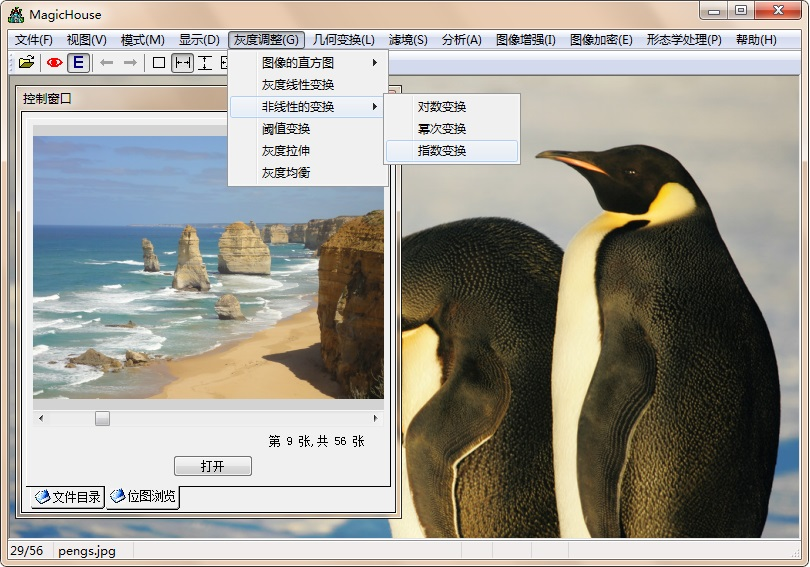纯C++，没有调用任何外部包，好处是一砖一瓦完全自我打造，坏处是我不能保证所有算法的效率都最优。目前该软件仍然只支持BMP和JPG两种格式（自己写编码函数的孩纸伤不起啊）。在浏览模式中程序提供了包括十种图片载入的方式：向下扫描、双重扫描、右侧飞入、双侧飞入、垂直百叶窗、水平百叶窗、垂直栅条、水平栅条、方格效果、落雨效果。下面是一些效果图（原图是西安青龙寺的樱花，博主拍摄）——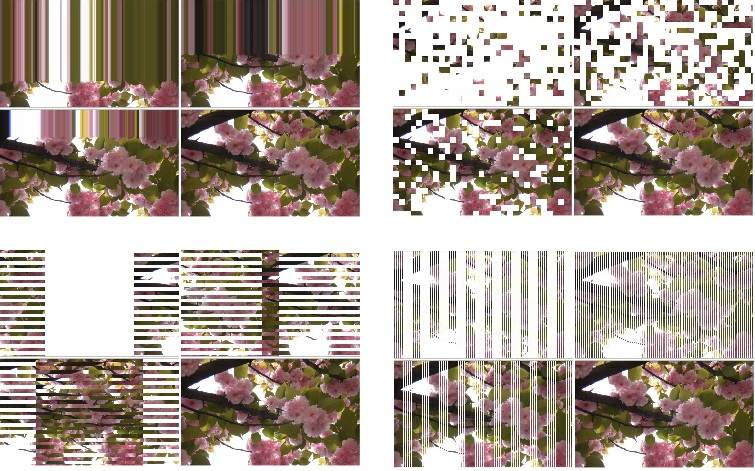下面是软件中实现了的部分图像处理算法演示：

图像的几何变换之旋转（双线性差值算法）（原图是广州大剧院，由博主的摄影师友人拍摄）→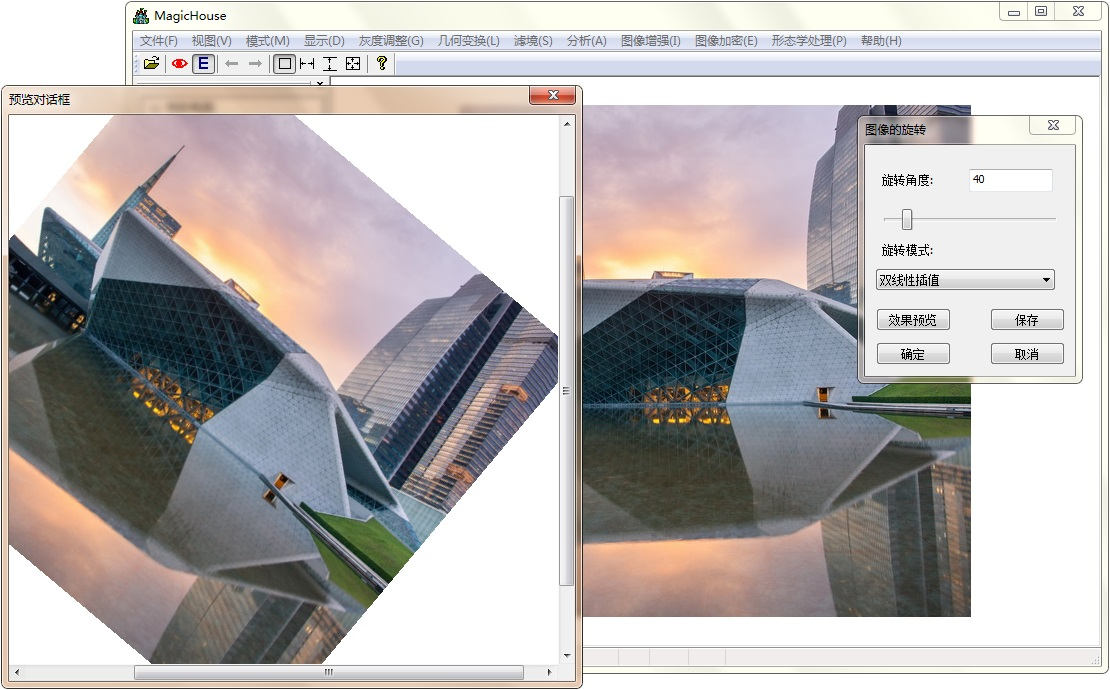图像的灰度变换之指数变换→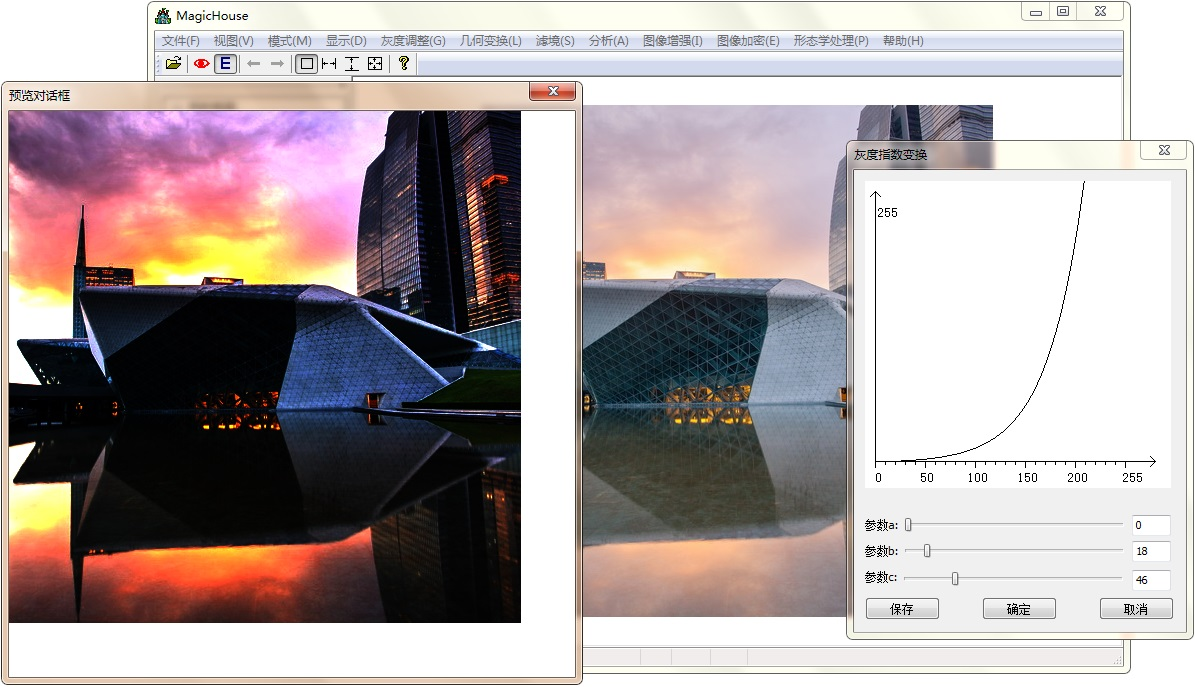图像滤镜之马赛克化（原图是广州南沙湿地，由博主拍摄）→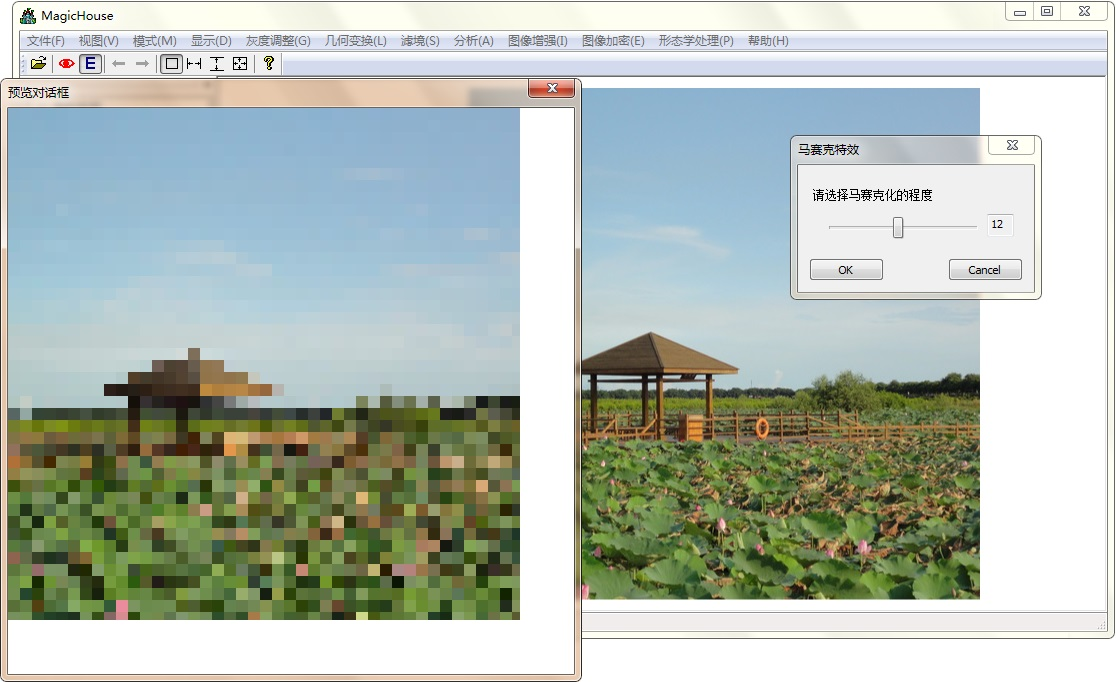图像滤镜之水雾效果（复合方向）→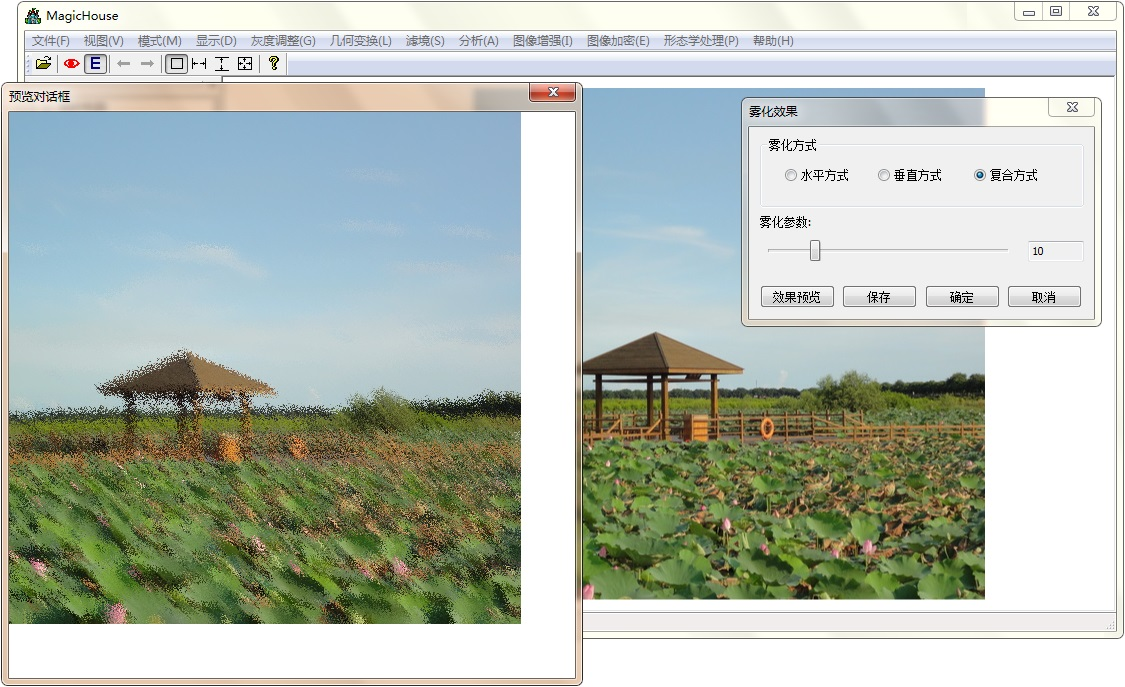图像滤镜之彩色钢笔效果→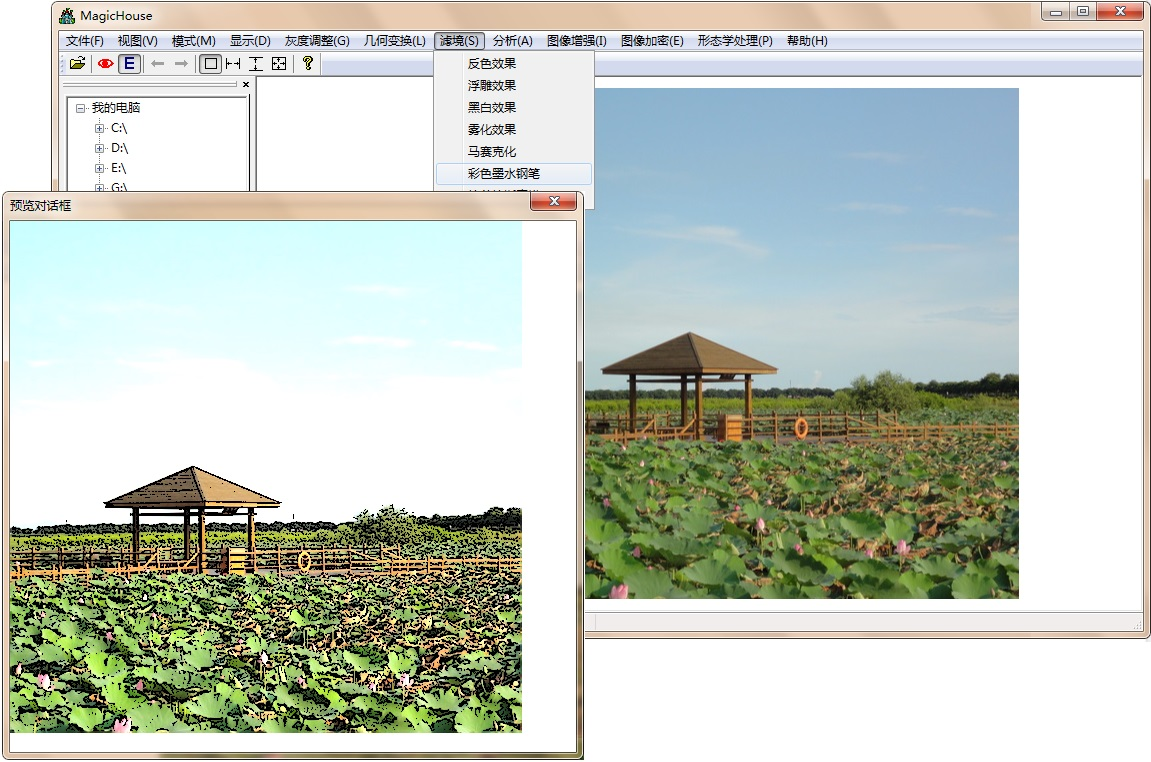图像分析之种子填充算法（原图是缅甸蒲甘的神庙，由博主拍摄）→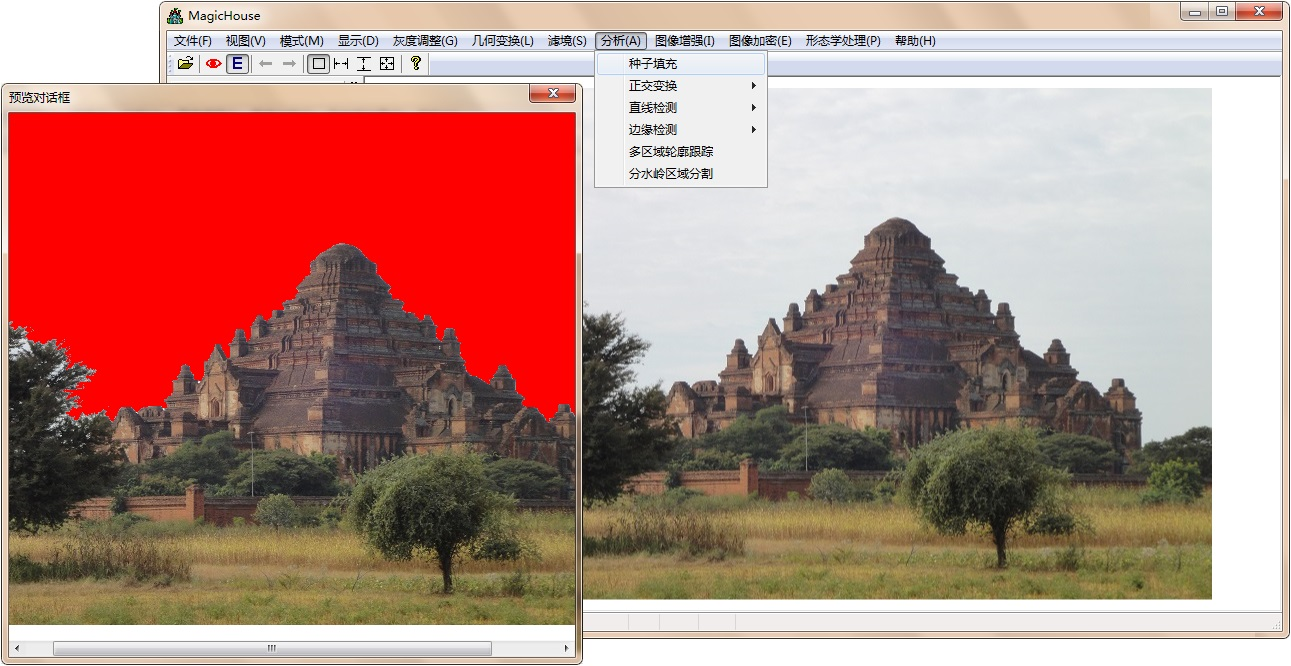正交变换之快速傅立叶变换→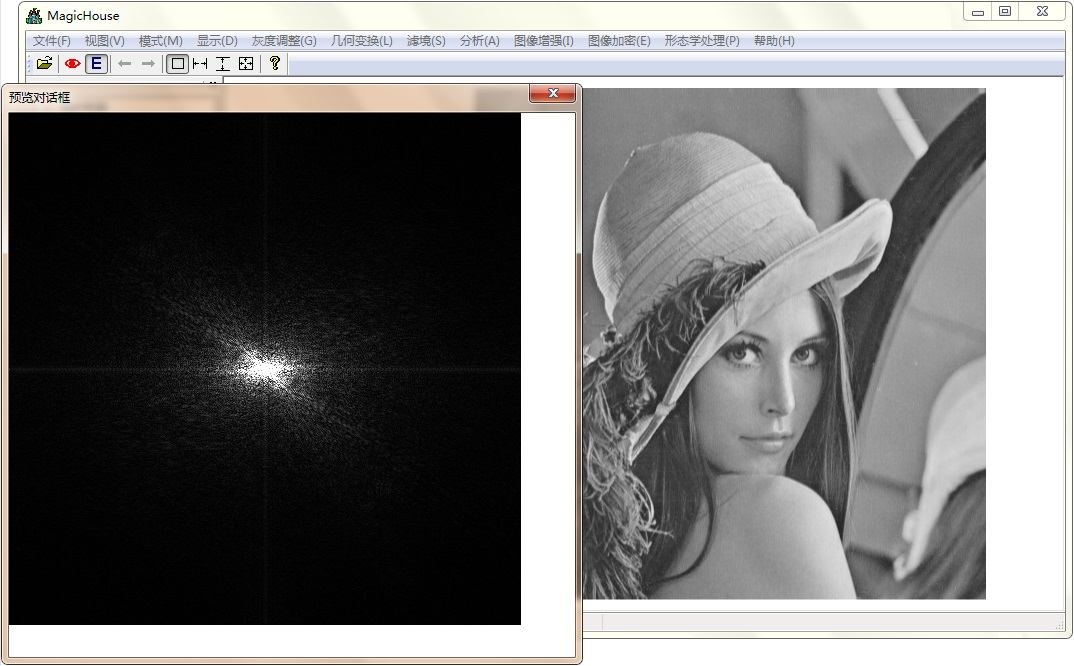正交变换之图像小波分解（二层）→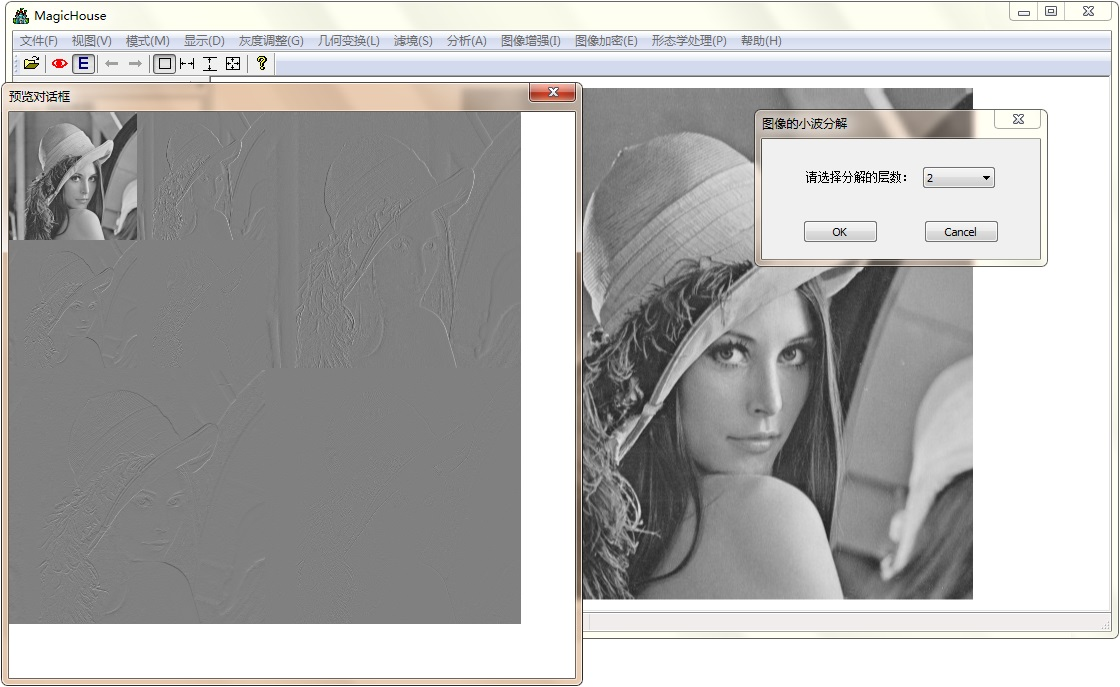基于Robinson算子的带方向的边缘检测→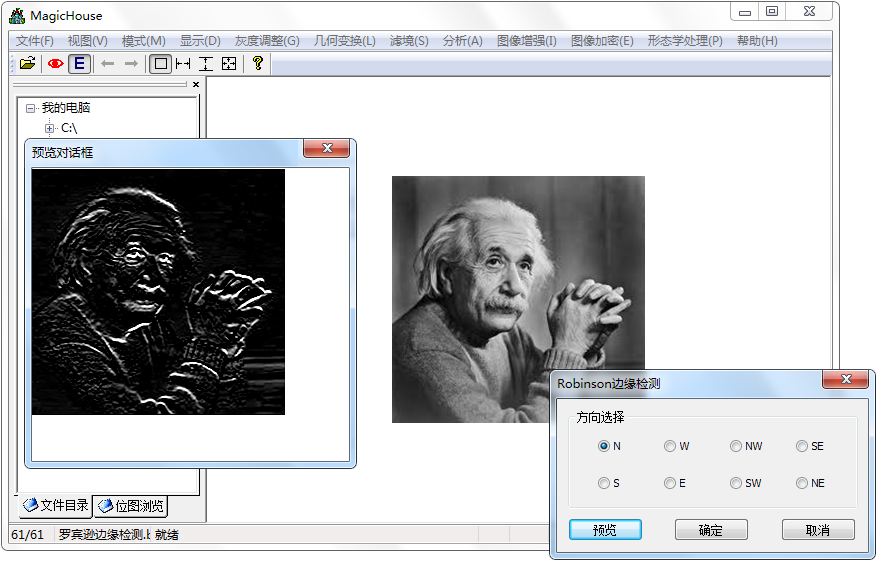多区域轮廓跟踪算法→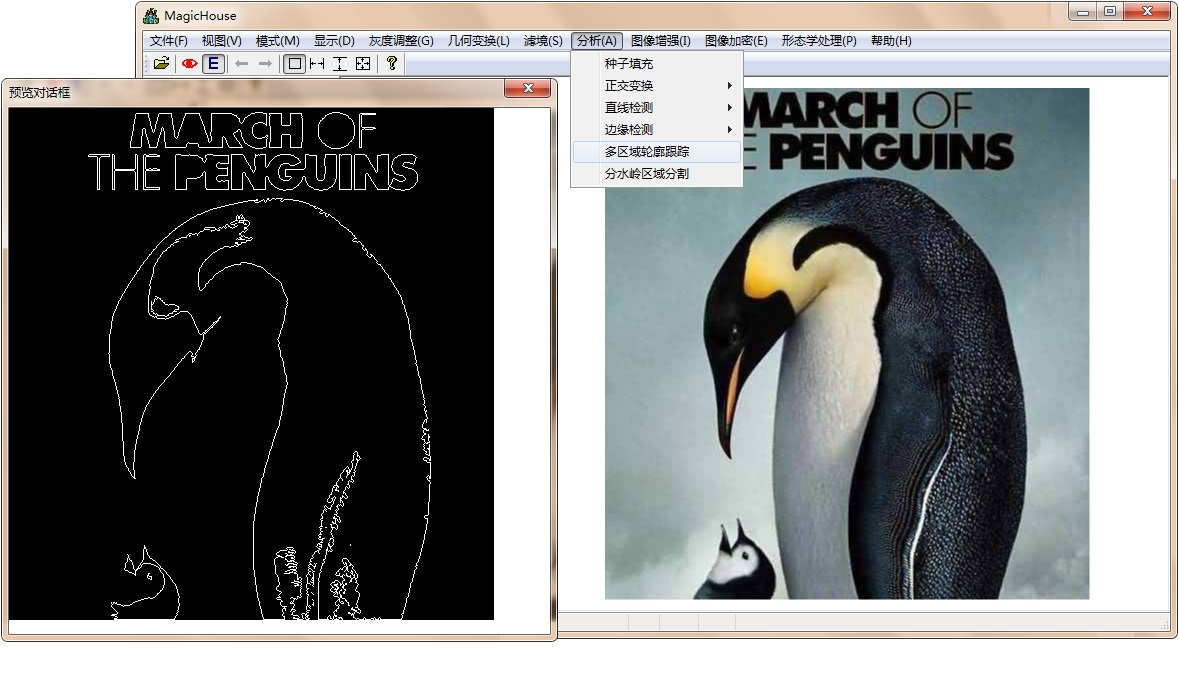图像分割之分水岭算法（原图是澳大利亚大洋路，由博主拍摄）→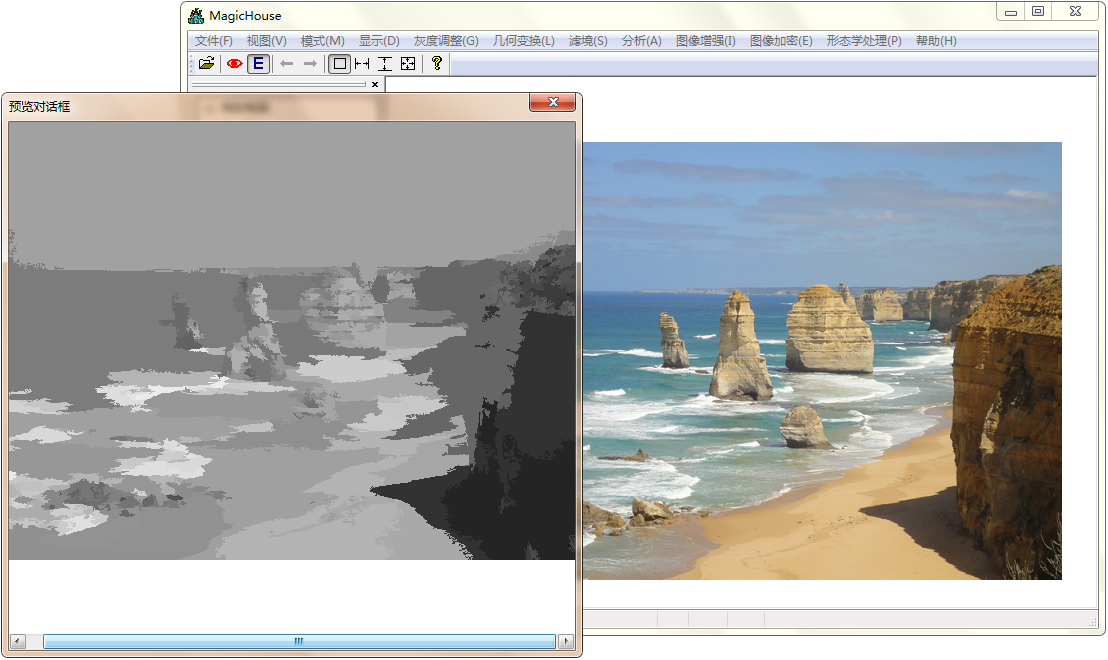图像增强处理之高斯平滑滤波→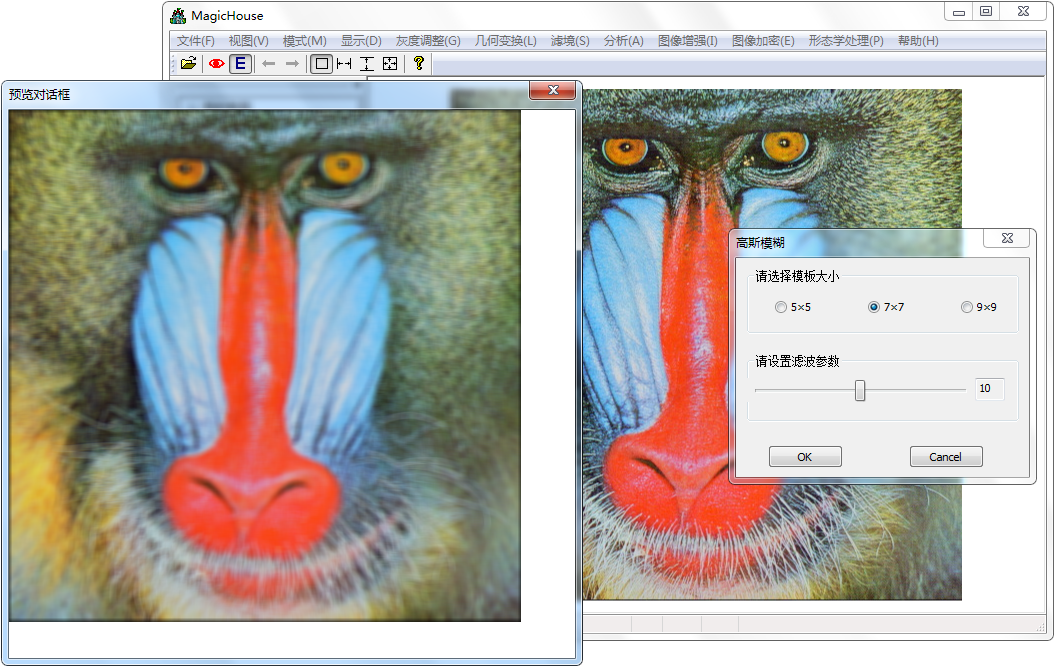采用中值滤波方法对受椒盐噪声污染的图像进行降噪→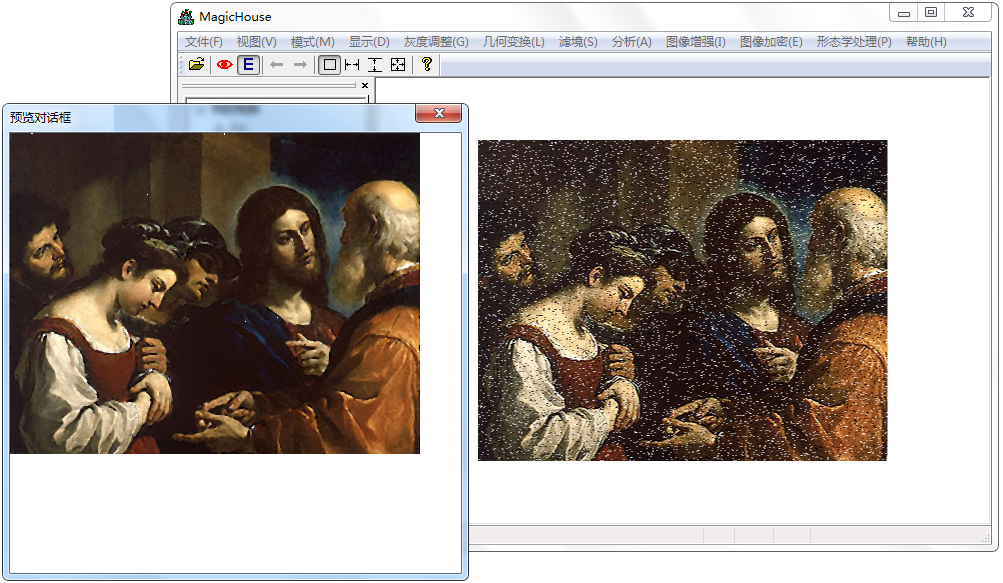高级图像增强处理之保护纹理的降噪算法：基于PM方程的非线性扩散滤波→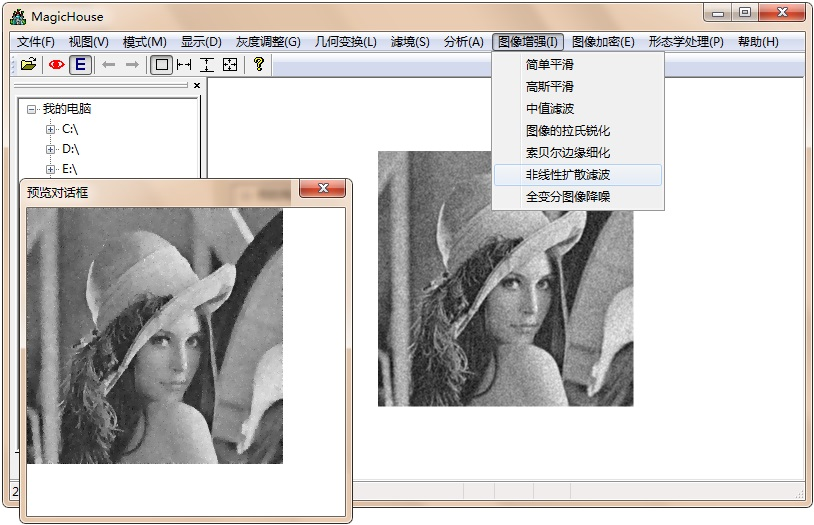高级图像增强处理之保护纹理的降噪算法：基于全变分的TV降噪算法→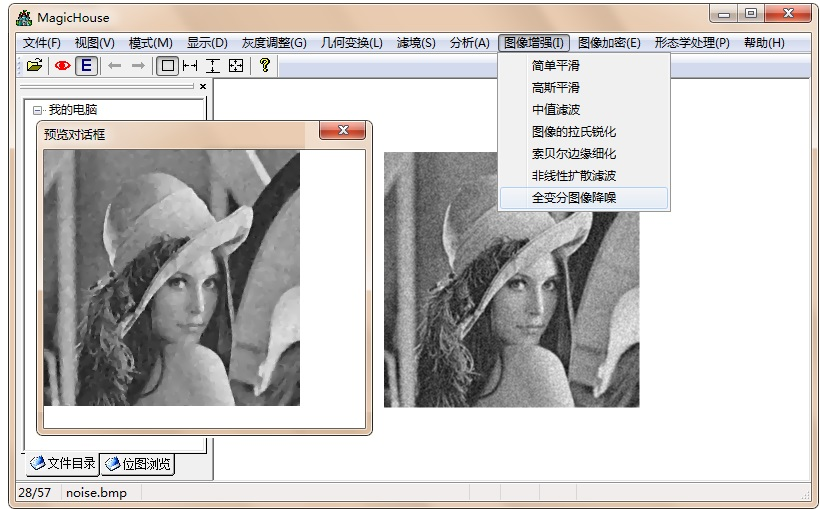图像加密算法之 基于Hilbert曲线的图像置乱加密（中图），以及基于Cat映射的图像置乱加密（右图）→

什么都看不到，要的就是这种效果：)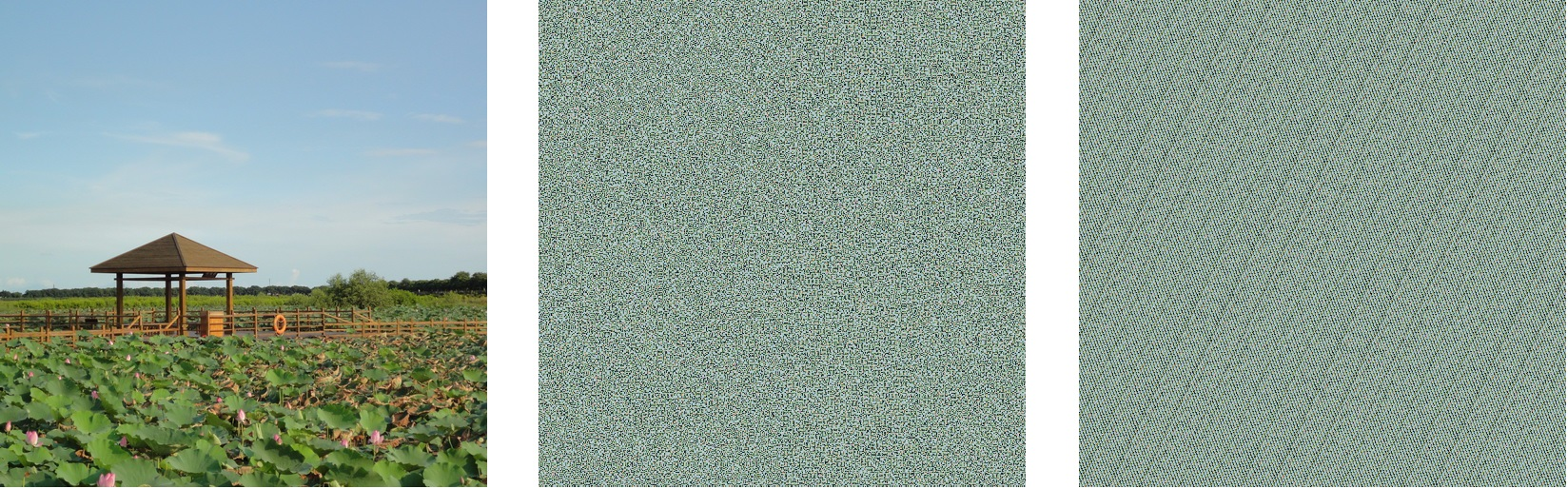基于混沌图像融合的密图隐藏算法→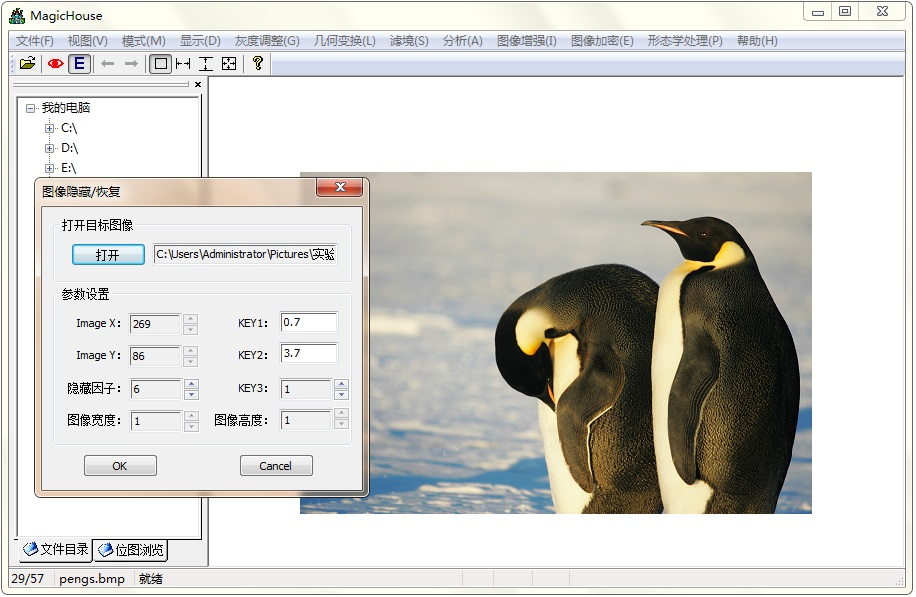下面的左图是隐藏处理后的密图（注意为了让读者看清楚里面隐藏了一幅图像，我特意调整参数让它显现出来，事实上是可以做到完全隐藏的，那样图像就会和原模板图像看起来完全一致了）。右图是解密后复原的图像。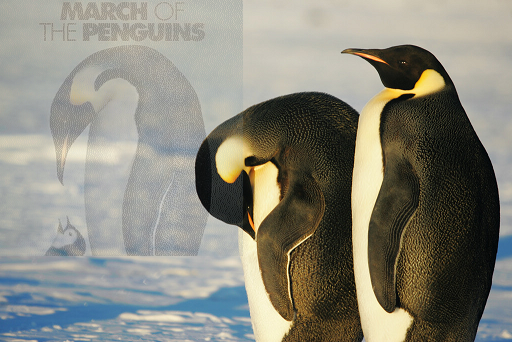基于形态学方法的图像处理之彩色图像的膨胀→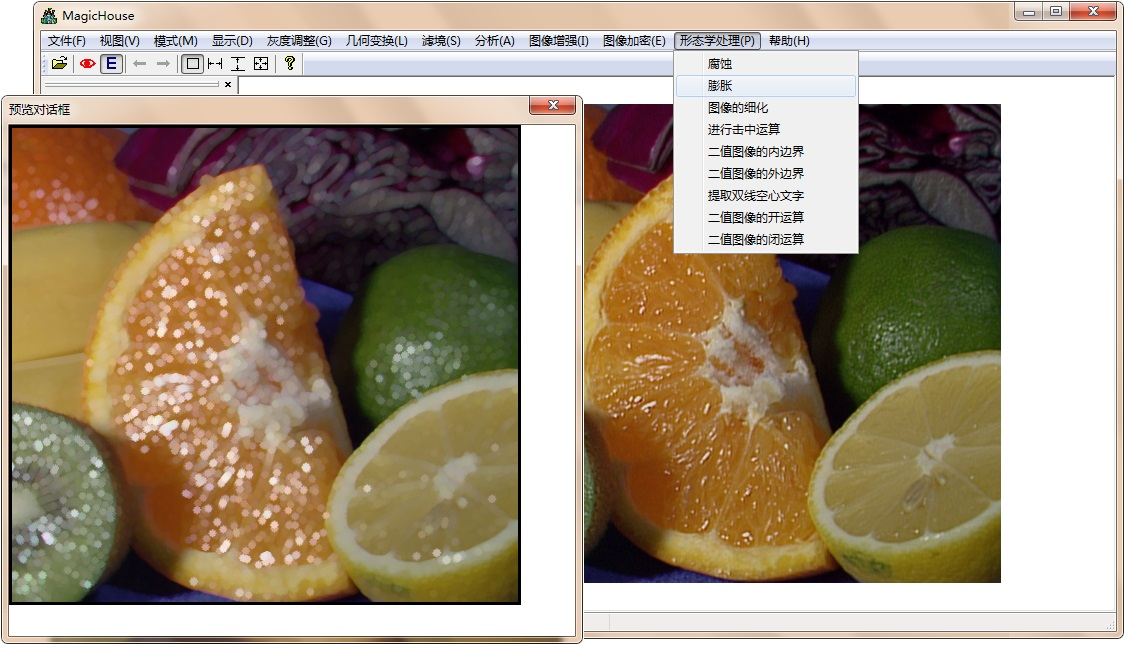以上列出的仅仅是软件中提供的部分功能，限于篇幅，这里就不逐一列出了，日后有时间我也会继续完善软件、实现更多有趣的算法。

现在提供最新（基于Visual Studio 2013）版本的程序下载，网盘链接：https://pan.baidu.com/s/1XaATmXllMFhByOJxCMsiZQ

此外，我还共享了我所使用的测试图片，因为1）部分算法对图像有特殊要求，比如某些形态学算法要求图像必须是二值图；2）因为犯懒，某些算法的parameter我采用了hard-coded的方式，所以最好用我提供的实验图像，以期得到理想的测试效果。


展开全文图像处理 算法
• 最近有人问我图像处理怎么研究，怎么入门，怎么应用，我竟一时语塞。仔细想想，自己也搞了两年图像方面的研究，个两个创新项目，发过两篇论文，也算是有点心得，于是总结总结和大家分享，希望能对大家有所帮助。在...


最近有人问我图像处理怎么研究，怎么入门，怎么应用，我竟一时语塞。仔细想想，自己也搞了两年图像方面的研究，做个两个创新项目，发过两篇论文，也算是有点心得，于是总结总结和大家分享，希望能对大家有所帮助。在写这篇教程之前我本想多弄点插图，让文章看起来花哨一点，后来我觉得没必要这样做，大家花时间沉下心来读读文字没什么不好，况且学术和技术本身也不是多么花哨的东西。

一、图像处理的应用

这个其实没什么好说的，一种技术的应用价值不是靠嘴上说，而是要看有多少人去搞，很简单的道理。其实我觉得判断一项技术有没有价值、有多大价值的最简单最有效的方法就是看有多少人在研究它。如果大家都在研究，那必然说明它很火，至少目前很火，以后的几年里依然还会火。因此，如果你不确定图像处理是不是有价值，那就查查全国图像处理工程师的数量就行了。

当然这里还是简单提一下。如果你真的只想问“图像处理有什么用？”，相信百度会给出比我更专业的答案。不过作为图像处理的行内人，我还是从几个基本的点来具体说一下。

1、身份认证

21世纪是刷脸的时代，这一点无可厚非。首先是银行，据说重庆的银行已经使用了人脸识别的验证系统来进行辅助的认证。其次是门禁系统，以前是指纹，虹膜，现在就是人脸。指纹、虹膜的识别虽然准确，但是有侵犯性，采集的过程中有侵犯性，验证的过程中也有侵犯性，反正让谁天天录指纹（采集指纹信息），用眼睛瞪摄像头（采集虹膜信息），谁都会觉得不舒服的，况且手还会脱皮。相比之下，人脸的识别就方便多了，拍张照片（采集人脸信息）谁都不会介意。最后是监控，一个摄像头所拍的监控能从不同的角度记录成百上千的人（比如车站等密集场所的监控），让警察去辨认将是一个浩大的工程，要是系统能够自动判别人员信息，无疑会给办案带来极大方便。

2、监控安防

安防监控可以说是图像处理领域最有潜力的应用领域。如今各个城市都在疯狂的安装监控摄像头，全国时刻都有无数的摄像头在录监控，但是安防监控的后端处理却没跟上。什么是后端处理，简单的说就是监控录像的视频处理。注意这里的视频处理可就不止包含人脸识别了，还有行人检测、异常检测、显著性检测、协同跟踪等。人脸识别之前说了，这里简单说说行人异常检测。图像处理中的行人异常检测在外行人眼中是一个非常有魔力的东西。毕竟让摄像头通过监控视频就能判断出当前画面中谁是好人谁是坏人（当然直接分为好人和坏人还是太武断了），在一般思维中貌似是不可能的。但是请不要忽略这样一个事实，就是目前大部分监控视频的分析处理都是由人工来完成的，警察破案时经常动不动就调出最近几天的监控视频，然后从头看到尾，其工程量之大可想而知。也正是这样才催生了人们对智能监控方面的研究，因为有这方面的实际需求。当然我们的视频分析程序不会直接给出诸如“好人or坏人”这样的武断而片面的判断结果。就当前的技术水平而言，能够统计当下监控画面中的人数（行人检测）、定位他们的人脸（人脸检测）、识别他们的身份（人脸识别）、判别他们的表情（表情识别）、检测他们的动作（异常检测），这就已经够了。这样以后人们就不会再面对长达几十甚至上百个小时的监控视频发呆，而是直接分析计算机给出的数据，当前画面中有多少人、都是谁、谁的动作可疑等。总之，接下来智能监控发展会很迅速，因为需求很迫切。

3、深度学习（Deep Learning）

通俗的说一句，“图像处理是深度学习应用的天堂”。深度学习这个概念可能有人不太熟悉，大家可以自行百度，我这里给出一个相对通俗的解释：“如果你不知道什么叫深度学习，就想想《终结者》中的T-800”。当然这句话不是我说的，是出自业界的一位大牛之口。当然这可能有点小片面，不过深度学习确实是公认的新一代人工智能的基础。

这里举两个例子。一是谷歌的人工大脑项目。谷歌公司可以说是深度学习方面的牵头企业了，其在2012年公布的谷歌大脑项目，动用了1.6万个计算节点，训练数周，得到的人工智能模型能够自主识别猫脸图像，为新一代人工智能开辟了道路，之后微软深度学习研究院、百度深度学习研究院等机构都开始大量投入，各个高校也搞得风声水起，原因很简单，大家都知道它会火。

第二就是图像识别方面的竞赛。最有权威的就是ImageNet竞赛。大家在一个拥有上千万张，上千类别的图像数据库上训练和测试自己的算法，比拼识别率。近几年来，摘得桂冠的一直都是深度学习模型，确切的说是卷积神经网络。更多有关ImageNet历年的竞赛信息大家可以自行百度。

说道深度学习在图像处理的应用，不得不提中国的汤晓鸥教授，说他是国内深度学习的领头羊也不为过。他提出的DeepID人脸识别算法（一共分为三代），在一些大规模人脸数据库上的正确率（若LFW库）已经达到了99.75%，单纯从数字上讲的话可以说已经超越了人类的识别率，为此汤教授还开办了公司，开发FaceSDK（虽然还没有公布）。不过拿计算机和人脑相比本身就是不合理的，各有所长嘛。不过可见DeepLearning在图像识别领域的强大威力。至于深度学习与图像处理的关系这里就不用多说了，谷歌大脑识别的是图像，深度学习竞赛用的是图像，DeepID识别的还是图像人脸，虽然深度学习在其他方面诸如语音识别等也有应用，在图像处理依然是其主要的应用领域。

二、图像处理研究工具

图像处理的研究分为算法研究和应用两个部分。用到的主要编程语言有Matlab、C/C++、Python等，原因很简单，它们都有很多相应的第三方库，不用我们从零开始编程。

1、Matlab

MathWork公司的Matlab软件可以说是算法研究的利器，它的强大之处在于其方便快捷的矩阵运算能力和图形仿真能力，单从简洁性和封装性来说，确实完爆其他语言。但高度封装必然会相应的损失一部分灵活性，况且Matlab严格的讲更像是一个工具，而非一门编程语言。顺便提一句，它在2015年编程语言排行榜中位于第20名，仅次于IOS开发的Objective-C。

对于算法研究人员（尤其是高校的硕士博士），首选工具自然是matlab，因为它简便快捷，封装性好，更重要的是全世界几乎所有的算法大牛、精英教授都会首先公布对应的Matlab源码，然后在逐步改写成其他语言进行实际应用。所以，如果你想做图像处理方面的研究，Matlab是必须掌握的，而且是熟练掌握。当你有一些想法需要验证时，最好明智的先用matlab编写出来测试。如果你上来就用看似高大上的C++来实验，不仅错误BUG一大堆，到头来可能效果还不佳，就算效果好，时间也会耽搁不少，毕竟算法开发还是要快的，这样才能赶在别人之前发论文。总之，只要是接触图像算法，终究逃不过Matlab，就算你是软件开发的，不研发算法，但总得能看懂别人的Matlab算法吧。

对于之前没怎么接触过Matlab与图像处理的人，在这里推荐一本相关的书籍《MATLAB图像处理实例详解（附光盘）》。这本书对于Matlab图像处理入门还是很有帮助的。记得我当时刚上研究生时就靠两本书入门的，一是冈萨雷斯的《数字图像处理》，二是这本《MATLAB图像处理实例详解》。不过这里友情提示，在看这类教程（不仅仅是Matlab）时千万不要试图去记忆所有的工具函数，这种做法是十分愚蠢的。正确的做法是根据自己的情况快速翻阅这类工具书，可以找出里面的有实际意义的源码来敲一敲练练手感，至于具体的工具函数，只需要知道Matlab提供了这方面的功能就行了，以后用到了再回来查，或者谷歌百度。我觉得在入门阶段，最重要的不是看了多少书，听了多少课，而是尽快自己敲出一段代码，运行出结果，来建立自信和成就感，这才是支持我们走下去的最实在的动力。记得我当时看了没多久就自己敲了一个蹩脚的车牌检测的Matlab程序，现在看来真是漏洞百出，不过当时我真的很兴奋，很有成就感，觉得自己能干这行，对于初学者来说，这种感受弥足珍贵。

2、OpenCv

Opencv是Intel公司开发的C++图像处理工具包，形象的理解为就是C++版的Matlab。当初Intel公司开发这个工具包的初衷也是方便大家共享，希望大家能够在一个共同架构的基础上共同建造摩天大楼，而不是各自在自己的地基上盖平房。与Matlab不同，Opencv是面向开发的，稳定性好，异常处理机制周全，但有一点需要注意，由于Opencv是开源的，那么如果你在项目中直接调用了它的API，那就意味着你的项目也必须开源。因此在真正的产品开发过程中，往往需要从Opencv库里面挖代码，而不是直接调用，幸好Intel公司允许我们看源码，自己编译一把就可以了。

说道C++和Opencv，有一个问题不得不提，那就是深度学习领域大名鼎鼎的Caffe框架。这是一个典型的基于C++和OpenCv的深度学习框架，由谷歌深度学习团队、“谷歌大脑”负责人之一贾扬清学者编写，并公布了源码。如今各个深度学习机构都在大量使用这个框架进行研究。

这里同样对推荐两本关于Opencv方面的教程。一本是CSDN博客大牛毛星云写的《OpenCV3编程入门》，这是它根据自己多年的博客整理成的书，很详细，很典型的一本教程，介绍了OpenCv中相对前沿的知识。我翻看过这本教程，中规中矩，里面的代码通俗易懂，尤其适合初学者。当然大家同样要注意不要犯了死读书的毛病，只看它的功能，敲代码练手感即可，不要试图记忆API函数。重要的工具用多了自然会记住，不重要的工具记住了也没用。

这里推荐的第二本书是《图像识别与项目实践――VC++、MATLAB技术实现》，这本书是一本偏向于工程应用的书，我之所以推荐它是因为它给出了很多有新意、能运行的代码。其中里面有一个项目让我印象很深，是一个车牌检测的实例。简单描述一下：由于车牌中的字符数是固定的，因此它通过判断横向区域笔画的跳变数以及笔画宽度来定位车牌区域。这个想法让人耳目一新，并且它还给出了详细代码，我也亲身试验过，效果还不错。

这里同样再强调一下，就是一定要尽早入手写程序，建立自信和成就感。我当时学OpenCv正好用它开发了一个人脸性别识别的系统，是一个本科大学生创新计划的需求，效果还可以。

3、Python

Python在今年12月份的编程语言排行榜中名列第5，增长迅速。可以说Python已经逐渐成为当下脚本语言的新标准。Python在图像处理算法方面除了其自身简洁的编程优势外，还得益于两个重要的Python类库——Numpy和Theano。

Numpy是Python的线性代数库，对于矩阵运算能提供很好的支持，并且能够在此基础上进行很多机器学习相关算法的开发仿真，这里推荐一本受到大家广泛认可的书《机器学习实战》，我最近也正在看这本书，里面对好多机器学习领域的经典算法，小到KNN，大到SVM，都给出了详细的介绍以及代码实现（Python版）。Theano是Python的机器学习库，能够方便的实现深度学习（例如卷积神经网络CNN）算法，网上很多对于DeepID算法的复现都是用的这个库。

人觉得单从图像处理的角度评价的话，Python并没有前面两个工具（Matlab和OpenCv）应用广泛，不过作为通用的脚本语言，我觉得每个程序员都应该去了解了解它，毕竟俗话说没有烂的编程语言，只有烂程序员。我在学Python时第一个自己写的程序就是微信打飞机的小程序，在我的博客中有详细的教程，虽然是参照小甲鱼的《零基础入门学习Python》视频教程写的，但还是蛮有成就感的。

三、图像处理研究方法

我觉得，图像处理研究主要可以分为三个部分：基础概念、基本思想、算法研究。

1、基础概念

所谓基础概念，就是图像处理里最基本的知识，比如什么是图像？什么是像素？什么是彩色图像等等。没有一个明确的界限来划定什么是基础概念什么是高级知识，因人而异。了解图像处理的基础知识，有一本书是必读的，就是冈萨雷斯编写的、阮秋琦翻译的《数字图像处理》。这本书已经作为图像处理领域的经典教材使用了三十多年，我自己也把这本书看了好几遍，每一遍都会有新的体会。我觉得每一个搞图像的都应该熟读这本书。书中除了有几章内容在讲小波变换、模式识别等相对抽象的内容外，其他内容相对都是很基础的，本科生水平就能看懂。而且我建议要尽早看这本书，如果是研究生尽量在进入课题之前就看一遍，因为这样一本经典的书在进入课题之后可能就没时间看了，以后也顶多是查阅而已。我当初就是在大四的寒假看完了这本书，以后在图像入门的过程中就显得轻松很多。看完这本书，哪怕是只看前几章，明白了什么是图像（二维或者三维矩阵）、什么是像素、彩色图和灰度图、颜色空间、图像滤波、图像噪声、图像频域变换等概念，将来在进行更深一步的研究的话就会方便很多了。

2、基本思想

刚开始想把这部分内容命名为“基本算法”，意在介绍图像处理中的一些基本算法，后来仔细想想决定不这么写，因为图像处理是一个非常大的概念，图像处理不等于人脸识别，也不等于模式识别，直接介绍诸如图像处理基本算法之类的内容很容易写成空话，没有什么实际意义。读者有兴趣的话可以直接谷歌百度“图像处理十大经典算法”，上面有我想说的内容。

万变不离其宗，算法是死的，重在思想。举个例子，我个人是主攻模式识别方向，在这个方向判断一个学生是否入门有一个非常简单的方法，就是“如果你能把图像很自然的想象成高维空间中的一个点”，那就说明在模式识别方面入门了，可以对图像进行分类了。当然标准不是唯一，在其他领域如目标检测也会有其他的判断标准，总之我们要对图像进行处理，那么图像就不再只是图像，它可能会演变成各种不同形式的概念，可能是点，可能是面，还可能是一个坐标空间。在目标跟踪的经典算法粒子滤波中，将一个个的小图像块看做一个个粒子；在子空间理论中，将一系列图像放在一起构建一个成分主空间（例如主成分分析PCA算法等等。，我不会详细介绍这些算法，说多了就显得抽象老套，但我要说的是我们一定要把图像本身理解好，它是一个图像，是一个矩阵，是一个信息的容器，是一种数据的表现形式，图像不一定都必须在视觉上有意义（比如频域的图像）。

总之图像处理的基本思想还是要立足于图像本身，要深度到图像内部结构中，思维要灵活。我当时做本科毕设时，怎么也不知道图像和高维空间中的点之间有什么对应关系，后来总算有一天，突然就明白了，这也就是所谓的量变产生质变。总之一定要多想，多总结，主动去钻研，才能够真正领悟一些东西。最基本的东西往往蕴藏着深奥的道理，无论你现在多牛多厉害，都不能放掉最本源的东西。多想想图像是什么，有什么本质属性，你可能无法得到准确的答案，但肯定能得到一些有用的感悟（有点像哲学问题了）。

3、算法研究

算法研究应该是图像处理的核心工作，尤其是各大高校的博士硕士。这里我并不想谈那些高大上的算法，我更想说的是一些算法研究的一些基础的东西，比如说一些基础课程，比如说矩阵运算。

研究图像处理的算法，离不开数学。在这里我建议图像处理方面的硕士一定要上两门课：《泛函分析》以及《最优化算法》，有的学校已经将这两门课列为了研究生阶段的必修课程。这两门可可以说是图像处理（至少是模式识别）的基础。我当初没上过最优化算法，但后来也自己补上了，不然真的是寸步难行。至于泛函我当时听课的时候也不是很懂，但是在之后的研究过程中发现很多图像处理的基本知识基本理论都和泛函分析中枯燥的定理如出一辙，没办法，有的东西本身就是枯燥的干货，学着费力，缺它不行。

其次我想说的是矩阵运算。图像就是矩阵，图像处理就是矩阵运算。大家为什么都喜欢用Matlab，就是因为它的矩阵运算能力实在是太强大，在Matlab的世界中任何变量都是矩阵。同样OpenCv之所以能流行，不仅仅是因为它良好的封装性，也是因为它的矩阵格式，它定义了Mat基础类，允许你对矩阵进行各种操作。Python也不例外，它的Numpy就是一个专门的线性代数库。

真正在图像编程过程中，那些看着高大上的API函数归根到底都是工具，查查手册就能找到，真正核心还是在算法，算法是由公式编写的，公式的单元是变量，而图像届的变量就是矩阵。所以，熟练去操作矩阵，求秩、求逆、最小二乘，求协方差，都是家常便饭。所以，如果你有幸能上《矩阵分析》这门课，一定要把它看懂，那里面都是干货。

四、小结

总之，图像处理就是一个典型的门槛低、厅堂深的领域。不需要太多基础，学过线性代数，会一点编程就够了；但是那些算法却深不可测，是个消耗功夫的活儿。在写这篇教程时我说的很直白，就像和大家对话一样，想到什么说什么。在最后我想说两句题外话，就是不仅仅针对图像处理，对于其他新技术的入门学习也是一样，尽快迈出第一步，尽快去建立自信和成就感，让自己有勇气走下去，然后缺什么补什么就行了。我觉得真正让人望而却步的往往不是技术本身，而是我们对自身的不自信。唯有果断开工，才能战胜心魔。


展开全文图像 python
• int main() {  img= imread("D:\\pic.jpg");//这里需要填写绝对路径。 imshow(img); getchar(); }
• 由于现在手头的学习是关于图像处理的，有些技能肯定迟早要上，于是决定利用假期学习Visual C++的相关知识。 今天是第一天，主要学习使用visual c++进行面向对象编程的基本方法。并给出数字图像处理基本类，以作为...
• 阅读提示： 《C++图像处理》系列以代码清晰，可读性为主，全部使用C++代码。 《Delphi图像处理》系列以效率为侧重点，一般代码为PASCAL，核心代码采用BASM。 尽可能保持二者内容一致，可相互对照。 本文代码必须...c C
• 用c++进行数字图像处理的时候一些基本的图像处理代码网络上哪里可以找到？
•   学习数字图像处理，首先我们就得对图像以及属性有一个直观的认识。记住这些概念，主要是为了方便跟同行业的人交流，以及阅读相关文献。 常见图像文件格式 BMP格式 BMP格式是windows系统下常用图像文件格式，...数字图像处理 图像的基本概念 图像深度 图像坐标系
• 本书全面系统地讨论了数字图像处理的理论、设计及应用。全书由自成体系而又互有联系的12 章组成，分别讨论了位图及图像类的概念、图像获娶图像增强、图像复原、正交变换、压缩编码、图像配准、运动检测、特征...图像处理 算法 文档 c
• “工欲善其事必先利其器”，使用一个优好的开发环境，能够快速入门。OpenCV环境配置
• 阅读提示： 《C++图像处理》系列以代码清晰，可读性为主，全部使用C++代码。 《Delphi图像处理》系列以效率为侧重点，一般代码为PASCAL，核心代码采用BASM。 尽可能保持二者内容一致，可相互对照。 本文代码必须...c C
• 阅读提示： 《C++图像处理》系列以代码清晰，可读性为主，全部使用C++代码。 《Delphi图像处理》系列以效率为侧重点，一般代码为PASCAL，核心代码采用BASM。 尽可能保持二者内容一致，可相互对照。 本文代码必须...c
• 在数字图像处理中，滤波是一个很重要的操作，许多算法其本质都是滤波操作。使用白话的形式对滤波定义：对于一个像素点，使用其领域像素（可以包含自身，也可不包含）的相关特性，计算出一个值，代替当前像素值。举个...均值滤波
• 阅读提示： 《C++图像处理》系列以代码清晰，可读性为主，全部使用C++代码。 《Delphi图像处理》系列以效率为侧重点，一般代码为PASCAL，核心代码采用BASM。 尽可能保持二者内容一致，可相互对照。 本文代码必须...c matrix transform
• 阅读提示： 《C++图像处理》系列以代码清晰，可读性为主，全部使用C++代码。 《Delphi图像处理》系列以效率为侧重点，一般代码为PASCAL，核心代码采用BASM。 尽可能保持二者内容一致，可相互对照。 本文代码必须...c C
• Python做图像处理 最近在一件比较 evil 的事情——验证码识别，以此来学习一些新的技能。因为我是初学，对图像处理方面就不太了解了，欲要利吾事，必先利吾器，既然只是一下实验，那 Python 来作原型开发再...python 图像处理 filter import image
• 基于Visual C++开发 左飞 万晋森 刘航 著 ISBN 978-7-121- 12776-2 2011年4月出版 定价：89.00元（含光盘1张） 16开 652 页 宣传语 《Visual C++数字图像处理开发入门...图像处理 编程 算法
• 图像处理算法1总结如下： //添加椒盐噪声 void salt(Mat&amp; src,int number) { for (int i = 0; i &lt; number; i++) { int r = static_cast&lt;int&gt;(rng.uniform(0, src.rows)); int ...图像处理算法
• <br />说来惭愧，我所在的是个图像处理课题组，可进组半年了，图像处理的理论知识还仅仅局限于王卫江老师的课。作业也一直是Matlab的。Matlab工具箱里的函数很全，直接调用很方便。可是速度不该恭维，尤其...图像处理 算法 matlab
...